• MATLAB中，使用遗传算法完成路径规划的问题，简单来说就是走迷宫。使用MATLAB进行仿真与实现。
• 遗传算法matlab代码实现路径规划，栅格法画地图，障碍物位置可以自己定义，画出平均路径和最短路径曲线，适应度函数考虑路线顺滑度和距离两个因素
• 本代码主要利用MATLAB工具实现MATLAB——遗传算法路径规划，简单明了，易于理解
• 遗传算法 网络图的路径规划 MATLAB代码可直接运行遗传算法 网络图的路径规划 MATLAB代码可直接运行遗传算法 网络图的路径规划 MATLAB代码可直接运行
• 采用栅格对机器人的工作...用遗传算法解决优化问题时的步骤是固定的，就是种群初始化，选择，交叉，变异，适应度计算这样，那么下面我就说一下遗传算法求栅格地图中机器人路径规划在每个步骤的问题、难点以及解决办法。
• 上述算法使用了连接线中点的条件，因此不是整个规划空间的最优路径，然后利用遗传算法对找到的最短路径各个路径点Pi (i=1,2,…n)调整，让各路径点在相应障碍物端点连线上滑动，利用Pi= Pi1+ti×(Pi2-Pi1)（ti∈[0,1]...
• 关于遗传算法的一个简单例子，在MATLAB中实现搜寻最短路径（最优化）的目的，仅供大家参考学习，谢谢
• MATLAB 实现基于遗传算法路径规划，只是一个9x9方格的小程序。
• 路径规划主要是让目标对象在规定范围内的区域内找到一条从起点到终点的无碰撞安全路径路径规划中有静态路径规划以及动态路径规划，本文所讨论的...本文采用遗传算法和模拟退火混合遗传算法进行求解，得到最优路径


文章目录
一、问题描述二、遗传算法设计2.1 算法原理2.2 编码2.2 适应度函数2.3 混合遗传算法
三、实验结果及分析四、总结参考文献MATLAB代码主程序相关函数

一、问题描述
路径规划主要是让目标对象在规定范围内的区域内找到一条从起点到终点的无碰撞安全路径。路径规划中有静态路径规划以及动态路径规划，本文所讨论的问题仅针对静态路径规划。具体问题描述如下： 给定起点、终点和障碍物等环境信息，如图1.1所示，利用演化计算方法得到最优轨迹，并分析不同参数选择对结果的影响。本文采用遗传算法和模拟退火混合遗传算法进行求解，得到最优路径。二、遗传算法设计
2.1 算法原理
遗传算法（Genetic Algorithm，GA）是进化计算的一部分，是模拟达尔文的遗传选择和自然淘汰的生物进化过程的计算模型，是一种通过模拟自然进化过程搜索最优解的方法。该算法简单、通用，鲁棒性强，适于并行处理。基本遗传算法流程包括种群初始化、选择、交叉、变异等操作，如图2.1所示。遗传算法的进化过程从完全随机生成的种群开始，随后逐代向适应度更高的种群进化。在进化的每一代中，整个种群的适应度按照一定规则被评价，基于个体的适应度高低从当前种群中随机选择多个个体，通过个体自然选择和基因突变操作而产生新种群，生成的新种群在算法的下一次迭代中成为当前种群。在遗传算法中，需解决的问题的解通常被成为基因个体，它通常以参数列表的形式进行标示，被称作染色体或基因串。其中染色体通常以编码形式体现，即表达为简单的字符串或数字串。
针对本问题，使用遗传算法求解最优路径需要按照特定规则初始化种群，并且根据问题所给的限定条件设计合适的适应度计算方法。下面的内容会详细说明这两个步骤，而算法中的其他操作，如选择、交叉、变异分别采用经典的轮盘赌、单点交叉和随机变异方法，这里不再赘述。
2.2 编码
针对两点间避障路径规划问题，考虑将种群中的每一个个体作为一条路径，个体由一系列坐标点

(

x

,

y

)

(x, y)

组成，所以个体的染色体长度为坐标点数。为了编程方便，这里将个体的坐标分开存储，得到两个种群

P

o

p

x

Popx

和

P

o

p

y

Popy

，在进化过程中将两个种群看作一个种群，共同优化。

P

o

p

x

i

=

(

x

1

,

x

2

,

…

,

x

j

)

,

P

o

p

y

i

=

(

y

1

,

y

2

,

…

,

y

j

)

.

Pop{x_i} = ({x_1},{x_2}, \ldots ,{x_j}),Pop{y_i} = ({y_1},{y_2}, \ldots ,{y_j}).

其中

P

o

p

x

i

Popx_i

和

P

o

p

y

i

Popy_i

表示种群中的第i个个体，

i

∈

[

1

,

M

]

i \in \left[ {1,M} \right]

；

x

j

x_j

和

y

j

y_j

为个体染色体中的一对编码，表示一个点的横纵坐标，

j

∈

[

1

,

N

]

j \in \left[ {1,N} \right]

；

M

M

和

N

N

分别表示种群数量和染色体长度。
2.2 适应度函数
适应度函数要有效反映每一个体与问题的最优个体之间的差距。遗传算法在进化搜索中基本不利用外部信息,仅以适应度函数为依据,利用种群中每个个体的适应度值来进行搜索。本问题的目标是路径总长度为最短，路径总长度的倒数就可以作为适应度函数：

F

i

t

n

e

s

s

=

1

∑

i

=

1

M

−

1

(

x

i

+

1

−

x

i

)

2

+

(

y

i

+

1

−

y

i

)

2

{\rm{ Fitness }} = \frac{1}{{\sum\limits_{i = 1}^{M - 1} {\sqrt {{{({x_{i + 1}} - {x_i})}^2} + {{({y_{i + 1}} - {y_i})}^2}} } }}

式中，

x

i

x_i

和

y

i

y_i

是种群中第i个个体的坐标；

M

M

为种群规模。
为了计算距离，需要判断点和两点连线与障碍物的关系，即判断个体染色体的

x

，

y

x，y

编码对是否在圆形障碍物内，以及相邻编码对所连成的线段是否与圆形障碍物有交点。若存在点

(

x

,

y

)

(x, y)

不满足上式的条件，则将该个体的适应度置零。

(

x

−

x

0

)

2

+

(

y

−

y

0

)

2

>

r

2

{(x - {x_0})^2} + {(y - {y_0})^2} > {r^2}

经过上一步的判断，符合条件的个体编码坐标点都在圆形障碍物外，所以这时判断相邻两坐标点组成的线段与障碍物的关系可分为如图2.2所示的三种情况讨论：在分情况讨论之前，还需要计算圆心到直线的距离

d

d

，这是为了区别圆心在直线上的特殊情况。计算公式为：

d

=

∣

A

x

0

+

B

y

0

+

C

∣

A

2

+

B

2

d = \frac{{\left| {A{x_0} + B{y_0} + C} \right|}}{{\sqrt {{A^2} + {B^2}} }}

对于第一种情况和第二种情况，

d

<

r

d<r

。计算

∠

O

A

B

\angle OAB

和

∠

O

B

A

\angle OBA

的余弦函数，若都大于0则该线段与圆相交，不符合条件，将该个体的适应度置零。计算公式为：

cos

⁡

∠

O

A

B

=

A

O

→

⋅

A

B

→

∣

A

O

→

∣

∣

A

B

→

∣

>

0

⇒

A

O

→

⋅

A

B

→

>

0

\cos \angle OAB = \frac{{\overrightarrow {AO} \cdot \overrightarrow {AB} }}{{\left| {\overrightarrow {AO} } \right|\left| {\overrightarrow {AB} } \right|}} > 0 \Rightarrow \overrightarrow {AO} \cdot \overrightarrow {AB} > 0

对于第三种情况，需要判断BA和BO的距离，

d

=

0

d=0

。若满足

∣

B

A

→

∣

>

∣

B

O

→

∣

\left| {\overrightarrow {BA} } \right| > \left| {\overrightarrow {BO} } \right|

，则证明该线段穿过圆，不符合条件，将该个体的适应度置零。
具体地，计算适应度函数的算法为：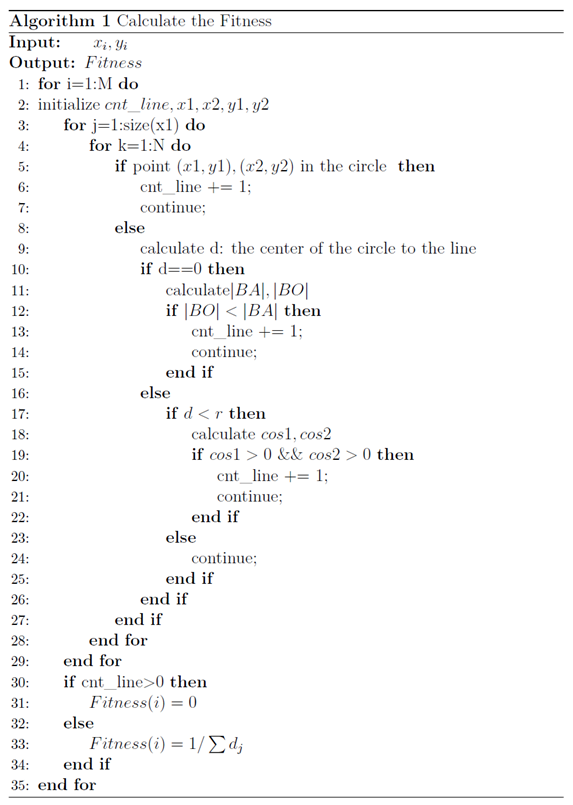2.3 混合遗传算法
为了提高遗传算法运行效率和求解质量，这里引入模拟退火算法的思想，模拟退火(Stimulated Annealing, SA)具有较强的局部寻优能力，并能使搜索过程避免陷入局部最优解[3-4]，采用模拟退火遗传算法（Stimulated Annealing Genetic Algorithm，SAGA）求解路径优化问题。
具体来说，模拟退火遗传算法是对适应度函数的改进，在前期（高温）减少个体间的适应度差异，后期（低温）放大个体间适应度差异，突出优秀个体，完成适应度拉伸的作用，在一定程度上弥补了遗传算法在前期容易早熟、后期容易停滞的缺点。具体的适应度值计算公式如式（6）和（7）所示：

f

i

=

e

f

i

T

∑

i

=

1

M

e

f

i

T

{f_i} = \frac{{{e^{\frac{{{f_i}}}{T}}}}}{{\sum\limits_{i = 1}^M {{e^{\frac{{{f_i}}}{T}}}} }}

T

=

T

0

(

A

g

−

1

)

T = {T_0}({A^{g - 1}})

其中，

M

M

为种群大小，

f

i

f_i

为第

i

i

个个体的适应度，

g

g

为遗传代数，

T

T

为温度，

T

0

T_0

为初始温度，

A

A

为退火速度（取0.99）。
三、实验结果及分析
本文实验环境为MATLAB2018b，探讨遗传算法的参数设置对结果的影响，比如迭代次数、交叉概率、变异概率，同时比较在相同参数条件下基本遗传算法和混合遗传算法的性能。最后通过可视化结果和路径距离长度来评估算法的准确性与有效性。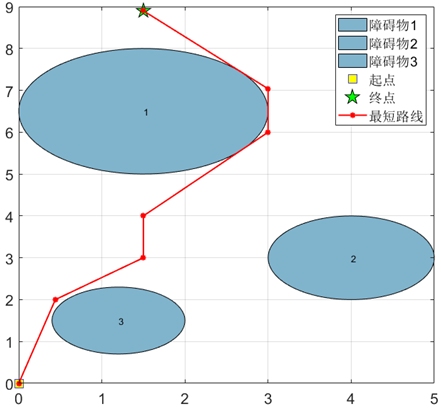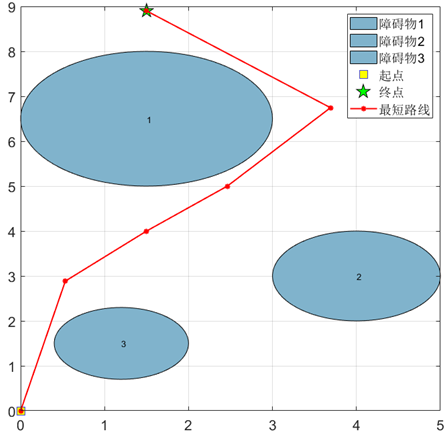四、总结
本次作业通过遗传算法编程求解了障碍物路径规划问题，对于基本遗传算法，探讨了算法中迭代次数、交叉概率和变异概率等参数对优化结果的影响。实验结果表明迭代次数不能设置得过小，否则算法还未收敛，反之则会导致计算资源的浪费；交叉概率和变异概率的大小影响种群的多样性，这两个参数过大会导致震荡，无法收敛，反之会导致优化出现停滞。此外，还将模拟退火的思想引入遗传算法，采用模拟退火遗传算法对本问题进行优化。实验结果证明GA算法更适用于该问题的优化求解，这是由于对该问题的个体坐标点排序和SAGA更强局部搜索能力导致无法使搜索过程进入最有希望的搜索区域共同造成的，同时SAGA较多的参数量也对算法寻优调参带来困难。
参考文献
 Holland J H. Genetic algorithms[J]. Scientific american, 1992, 267(1): 66-73.  王小平, 曹立明. 遗传算法——理论、应用与软件实现[M]. 西安交通大学出版社, 2002.  Kirkpatrick S, Gelatt C D, Vecchi M P. Optimization by simulated annealing[J]. science, 1983, 220(4598): 671-680.  王雪梅,王义和.模拟退火算法与遗传算法的结合[J].计算机学报,1997(04):381-384.  Sen M K, Datta-Gupta A, Stoffa P L, et al. Stochastic reservoir modeling using simulated annealing and genetic algorithm[J]. SPE Formation Evaluation, 1995, 10(01): 49-56.
MATLAB代码
主程序
GA_main.m
%%%%%%基本遗传算法(GA)%%%%%%%%%%%

clear
%%%设置超参数
p_crs = 0.7;   %交叉概率
p_mut = 0.1;   %变异概率
ratio = 0.5;   %选择操作中父辈的比例
pop_num = 5000;  %种群规模
chrom_len = 7;  %染色体长度，这里代表路线的点数
iteration = 40;

% 一个个体就是一条路线
[x,y]=popinit(pop_num,chrom_len);   %产生初始种群
fit=fitness(x,y);      %计算种群适应度
[bestx0,besty0,fit0]=best(x,y,fit);

for i=1:1:iteration           %设置进化代数
[Parentx,Parenty]=select(x, y, fit, ratio);      %选择
[Kidx,Kidy]=crossover(Parentx,Parenty,p_crs);       %交叉
[Kidx,Kidy]=mutation(Kidx,Kidy,p_mut);              %变异
x = [Parentx; Kidx];    % 得到新的种群
y = [Parentx; Kidy];
x(:,chrom_len)=1.5;  %保留终点
y(:,chrom_len)=8.9;

fit = fitness(x,y);   % 计算进化后的适应度
[bestx,besty,bestfit]=best(x,y,fit);   %选择每一代中的最佳个体
route_x(i,:)=bestx;                     %保存该最佳个体
route_y(i,:)=besty;
route_fit(i)=bestfit;
fprintf('%dth 代进化完成...\n', i)
%     plot(bestx,besty,'r-');
end
route_fit = [fit0, route_fit];     %加上初始种群中最优个体
route_x = [bestx0; route_x];
route_y = [besty0; route_y];

[final_fit,idx]=max(route_fit);            %所有代中的的最佳路线
final_routex=route_x(idx,:);
final_routey=route_y(idx,:);
final_distance = 1.0/final_fit             %最佳路径长度

%==========画图，可视化路线、进化过程==============
% start point
xs=0;
ys=0;
% Destination
xt=1.5;
yt=8.9;
%obstacle
xobs=[1.5 4.0 1.2];
yobs=[6.5 3.0 1.5];
robs=[1.5 1.0 0.8];
theta=linspace(0,2*pi,100);
max_area = 0;
for k=1:numel(xobs)
fill(xobs(k)+robs(k)*cos(theta),yobs(k)+robs(k)*sin(theta),[0.5 0.7 0.8]);  % 后一个参数表示RGB值
text(xobs(k), yobs(k), num2str(k))
hold on;
end
plot(xs,ys,'bs','MarkerSize',12,'MarkerFaceColor','y');
plot(xt,yt,'kp','MarkerSize',16,'MarkerFaceColor','g');
grid on;
hold on;

%%画出最短路径的路线
plot(final_routex,final_routey,'r*-',  'linewidth', 1.5);
legend('障碍物1','障碍物2','障碍物3','起点', '终点', '最短路线')
set(gca,'FontSize',16);
hold off;

% 进化过程中适应度曲线
figure,
plot(0:1:size(route_fit,2)-1, route_fit, 'linewidth', 1.2)
ylim([0.08,0.1])
title(['变异率=',num2str(p_mut),',交叉率=', num2str(p_crs), '的进化曲线']);
legend('适应度值', 'Location', 'southeast');
set(gca,'FontSize',16);

figure,
plot(1./route_fit, 'linewidth', 1.2)
title(['变异率=',num2str(p_mut),',交叉率=', num2str(p_crs), '的进化曲线']);
legend('最短路径长度值', 'Location', 'northeast');
set(gca,'FontSize',16);

% d_ga = 1./route_fit;
% save('d_ga');


SAGA_main.m
%%%%%%模拟退火遗传算法(SAGA)%%%%%%%%%%%

clear
%%%设置超参数
p_crs = 0.7;   %交叉概率
p_mut = 0.1;   %变异概率
ratio = 0.5;   %选择操作中父辈的比例
pop_num = 5000;  %种群规模
chrom_len = 7;  %染色体长度，这里代表路线的点数
iteration = 40;
T0 = 100;  %初始温度
A = 0.8;  %退火速度

% 一个个体就是一条路线
[x,y]=popinit(pop_num,chrom_len);   %产生初始种群
fit=saga_fitness(x,y, T0);      %计算种群适应度
[bestx0,besty0,fit0]=best(x,y,fit);
d0 = 0; %初始路径长度
for j=1:1:size(bestx0,2)-1
d0 = d0 + sqrt((bestx0(1,j+1)-bestx0(1,j)).^2 + ...
(besty0(1,j+1)-besty0(1,j)).^2);     %该个体（即路线）的路径长度
end

for i=1:1:iteration           %设置进化代数
[Parentx,Parenty]=select(x, y, fit, ratio);      %选择
[Kidx,Kidy]=crossover(Parentx,Parenty,p_crs);       %交叉
[Kidx,Kidy]=mutation(Kidx,Kidy,p_mut);              %变异
x = [Parentx; Kidx];    % 得到新的种群
y = [Parentx; Kidy];
x(:,chrom_len)=1.5;   % 保留终点
y(:,chrom_len)=8.9;

T = T0 * A^(i-1);  % 当前温度
fit = saga_fitness(x,y,T);   % 计算进化后的适应度
[bestx,besty,bestfit]=best(x,y,fit);   %选择每一代中的最佳个体
route_x(i,:)=bestx;                     %保存该最佳个体
route_y(i,:)=besty;
route_fit(i)=bestfit;
for j=1:1:size(bestx,2)-1
dd(j)=sqrt((bestx(1,j+1)-bestx(1,j)).^2 + ...
(besty(1,j+1)-besty(1,j)).^2);     %该个体（即路线）的路径长度
end
d(i) = sum(dd);   %有问题
fprintf('%dth 代进化完成...\n', i)
%     plot(bestx,besty,'r-');
end
route_fit = [fit0, route_fit];   %加上初始种群中最优个体
route_x = [bestx0; route_x];
route_y = [bestx0; route_y];
d = [d0, d];

[final_fit,idx]=max(route_fit);            %所有代中的的最佳路线
final_routex=route_x(idx,:);
final_routey=route_y(idx,:);
final_distance = min(d)             %最佳路径长度

%==========画图，可视化路线、进化过程==============
% start point
xs=0;
ys=0;
% Destination
xt=1.5;
yt=8.9;
%obstacle
xobs=[1.5 4.0 1.2];
yobs=[6.5 3.0 1.5];
robs=[1.5 1.0 0.8];
theta=linspace(0,2*pi,100);
max_area = 0;
for k=1:numel(xobs)
fill(xobs(k)+robs(k)*cos(theta),yobs(k)+robs(k)*sin(theta),[0.5 0.7 0.8]);  % 后一个参数表示RGB值
text(xobs(k), yobs(k), num2str(k))
hold on;
end
plot(xs,ys,'bs','MarkerSize',12,'MarkerFaceColor','y');
plot(xt,yt,'kp','MarkerSize',16,'MarkerFaceColor','g');
grid on;
hold on;

%%画出最短路径的路线
plot(final_routex,final_routey,'r*-',  'linewidth', 1.5);
legend('障碍物1','障碍物2','障碍物3','起点', '终点', '最短路线')
set(gca,'FontSize',16);
hold off;

% 进化过程中适应度曲线
figure,
% plot(0:1:size(route_fit,2)-1, route_fit, 'linewidth', 1.2)
plot(d, 'linewidth', 1.2)
% ylim([0.08,0.1])
title(['变异率=',num2str(p_mut),',交叉率=', num2str(p_crs), '的进化曲线']);
legend('最短路径长度值', 'Location', 'northeast');
set(gca,'FontSize',16);

d_saga = d;
save('d_saga');

figure,
plot(d, 'linewidth', 1.2), hold on,
plot(d_ga, 'linewidth', 1.2);
title(['变异率=',num2str(p_mut),',交叉率=', num2str(p_crs), '的进化曲线']);
legend('SAGA最优路径值', 'GA最优路径值', 'Location', 'northeast');
set(gca,'FontSize',16);


相关函数
best.m
%====================================
%%输入参数：种群、其适应度
%%输出参数：种群中的最佳个体，及其适应度
%%说明：
%     选择最佳个体，便于后续分析
%====================================
function [bestx,besty,bestfit]=best(x,y,fitval)
bestx = x(1,:);
besty = y(1,:);
bestfit = fitval(1);
for i = 2:size(x,1)
if fitval(i) > bestfit
bestx = x(i,:);
besty = y(i,:);
bestfit=fitval(i);
end
end
end

crossover.m
%====================================
%%输入参数：父代种群，交叉概率
%%输出参数：子代种群
%%说明：
%     单点交叉，最后需要从小到大排序
%====================================
function [kidx, kidy]=crossover(x, y, p)
[m,n] = size(x);
kidx = ones(size(x));
kidy = ones(size(y));
for i = 1:2:m-1
if(rand < p)
point = round(rand*n);
kidx(i,:) = [x(i,1:point),x(i+1,point+1:n)];
kidx(i+1,:) = [x(i+1,1:point),x(i,point+1:n)];
kidy(i,:) = [y(i,1:point),y(i+1,point+1:n)];
kidy(i+1,:) = [y(i+1,1:point),y(i,point+1:n)];
else
kidx(i,:) = x(i,:);
kidx(i+1,:) = x(i+1,:);
kidy(i,:) = y(i,:);
kidy(i+1,:) = y(i+1,:);
end
end
for i = 1:1:m
kidx(i,:) = sort(kidx(i,:));
kidy(i,:) = sort(kidy(i,:));
end
end

fitness.m
%====================================
%%输入参数：种群——x,y横纵坐标
%%输出参数：种群中每个个体的适应度值
%%说明：
%     逐个计算种群中个体的适应度值，判断该个体所连成线路与圆形障碍物之间的关系，
%     将满足条件的路径的距离倒数作为适应度值
%====================================

function fitval=fitness(x,y)
%obstacle
xobs=[1.5 4.0 1.2];
yobs=[6.5 3.0 1.5];
robs=[1.5 1.0 0.8];
[n, xn] = size(x);
for i=1:1:n
cnt_line = 0;  %记录穿过障碍物的线段数

%%拆分相邻的两个点，便于后续计算
for m=1:1:xn-1
x1(m)=x(i,m);
y1(m)=y(i,m);
end
for n=2:1:xn
x2(n-1)=x(i,n);
y2(n-1)=y(i,n);
end

%%判断线段与障碍物的关系
for m = 1:size(x1,2)
A = y2(m) - y1(m);
B = x1(m) - x2(m);
C = y1(m)*(x2(m)-x1(m)) - x1(m)*(y2(m)-y1(m));
for ii = 1:3
if ((x1(m)-xobs(ii))^2 + (y1(m)-yobs(ii))^2) < robs(ii)^2 || ((x2(m)-xobs(ii))^2 + (y2(m)-yobs(ii))^2) < robs(ii)^2
% disp('有点在圆内')
cnt_line = cnt_line + 1;
continue;
else
d = abs(A*xobs(ii)+B*yobs(ii)+C) / sqrt(A^2+B^2);
if d==0
d1 = (xobs(ii)-x1(m))^2 + (yobs(ii)-y1(m))^2;
d2 = (x2(m)-x1(m))^2 + (y2(m)-y1(m))^2;
if d1 < d2
cnt_line = cnt_line + 1;   % 该线段过圆心且穿过圆
end

elseif d < robs(ii)
cos1 = (x2(m)-x1(m))*(xobs(ii)-x1(m)) + (y2(m)-y1(m))*(yobs(ii)-y1(m));
cos2 = (x1(m)-x2(m))*(xobs(ii)-x2(m)) + (y1(m)-y2(m))*(yobs(ii)-y2(m));
if cos1>0 && cos2>0
cnt_line = cnt_line + 1;    % 该线段与圆交与两点
end
else
continue;
end
end
end

end

%%%计算适应度
if cnt_line > 0
f(i)=0;       %路线穿过障碍物的个体适应度置零
else
for j=1:1:xn-1
d(j)=sqrt((x(i,j+1)-x(i,j)).^2+(y(i,j+1)-y(i,j)).^2);     %该个体（即路线）的路径长度
end
f(i)=1.0/sum(d);       %取距离的倒数作为个体适应度
end
record_cnt(i,:) = cnt_line;
end
fitval=f';
%     disp(['不满足条件的最小线段数为：',num2str(min(record_cnt, [], 1))])

end


mutation.m
%====================================
%%输入参数：父代种群，变异概率
%%输出参数：子代种群
%%说明：
%     随机替换，最后需要从小到大排序
%====================================
function [kidx, kidy]=mutation(x, y, p)
[m, n] = size(x);
kidx = x;
kidy = y;
for i = 1:1:m
if(rand < p)
point = round(rand*n);
% 避免越界
if point <= 1
point = 2;
end
if point == n
point = n-1;
end
% 变异：随机数替换
kidx(i,point) = round(rand*n);
kidy(i,point) = round(rand*n);
end
end
for i = 1:1:m
kidx(i,:) = sort(kidx(i,:));
kidy(i,:) = sort(kidy(i,:));
end
end

popint.m
%====================================
%%输入参数：种群数量，染色体长度
%%输出参数：初始种群
%%说明：
%     种群中的个体由一系列点组成，该点的x，y坐标分开存放，
%     除了起点和终点，其余点随机生成并从小到大排序
%====================================
function [x,y]=popinit(popsize,chromlength)

x=5.0*rand(popsize,chromlength);
y=8.9*rand(popsize,chromlength);
x(:,1)=0;  % 设置起点
y(:,1)=0;

for i=1:1:size(x,1)
x(i,:)=sort(x(i,:));
y(i,:)=sort(y(i,:));
end

x(:,chromlength)=1.5;  %设置终点
y(:,chromlength)=8.9;

end

saga_fitness.m
%====================================
%%输入参数：种群——x,y横纵坐标, 当前温度T
%%输出参数：种群中每个个体的适应度值
%%说明：
%     逐个计算种群中个体的适应度值，判断该个体所连成线路与圆形障碍物之间的关系，
%     将满足条件的路径的距离倒数作为适应度值
%====================================

function fitval=saga_fitness(x,y,T)
%obstacle
xobs=[1.5 4.0 1.2];
yobs=[6.5 3.0 1.5];
robs=[1.5 1.0 0.8];
[n, xn] = size(x);
for i=1:1:n
cnt_line = 0;  %记录穿过障碍物的线段数

%%拆分相邻的两个点，便于后续计算
for m=1:1:xn-1
x1(m)=x(i,m);
y1(m)=y(i,m);
end
for n=2:1:xn
x2(n-1)=x(i,n);
y2(n-1)=y(i,n);
end

%%判断线段与障碍物的关系
for m = 1:size(x1,2)
A = y2(m) - y1(m);
B = x1(m) - x2(m);
C = y1(m)*(x2(m)-x1(m)) - x1(m)*(y2(m)-y1(m));
for ii = 1:3
if ((x1(m)-xobs(ii))^2 + (y1(m)-yobs(ii))^2) < robs(ii)^2 || ((x2(m)-xobs(ii))^2 + (y2(m)-yobs(ii))^2) < robs(ii)^2
% disp('有点在圆内')
cnt_line = cnt_line + 1;
continue;
else
d = abs(A*xobs(ii)+B*yobs(ii)+C) / sqrt(A^2+B^2);
if d==0
d1 = (xobs(ii)-x1(m))^2 + (yobs(ii)-y1(m))^2;
d2 = (x2(m)-x1(m))^2 + (y2(m)-y1(m))^2;
if d1 < d2
cnt_line = cnt_line + 1;   % 该线段过圆心且穿过圆
end

elseif d < robs(ii)
cos1 = (x2(m)-x1(m))*(xobs(ii)-x1(m)) + (y2(m)-y1(m))*(yobs(ii)-y1(m));
cos2 = (x1(m)-x2(m))*(xobs(ii)-x2(m)) + (y1(m)-y2(m))*(yobs(ii)-y2(m));
if cos1>0 && cos2>0
cnt_line = cnt_line + 1;    % 该线段与圆交与两点
end
else
continue;
end
end
end

end

%%%计算适应度
if cnt_line > 0
f(i)=0;       %路线穿过障碍物的个体适应度置零
else
for j=1:1:xn-1
d(j)=sqrt((x(i,j+1)-x(i,j)).^2+(y(i,j+1)-y(i,j)).^2);     %该个体（即路线）的路径长度
end
f(i)=1.0/sum(d);       %取距离的倒数作为个体适应度
end
record_cnt(i,:) = cnt_line;
end

I = find(f ~= 0);
sumf = sum(exp(f(I) ./ T)) + n - size(I, 1);
f(I) = exp(f(I)./T) ./ sumf;
fitval=f';
%     disp(['不满足条件的最小线段数为：',num2str(min(record_cnt, [], 1))])

end

select.m
%====================================
%%输入参数：种群、其适应度、选择比例
%%输出参数：父辈种群——x,y横纵坐标
%%说明：
%     按照输入参数的比例，从种群中选择父代
%====================================
function [parentPopx, parentPopy]=select(popx, popy, fitness, ratio)
totalfit=sum(fitness); %适应值之和
accP=cumsum(fitness/totalfit); %概率累计和

%轮盘赌选择算法
for n=1:round(ratio*size(popx,1))
mat=find(accP>rand); %找到比随机数大的累积概率
if isempty(mat)
continue
end

parentPopx(n,:)=popx(mat(1),:);%将首个比随机数大的累积概率的位置的个体遗传下去
parentPopy(n,:)=popy(mat(1),:);
end
end


展开全文模拟退火 计算智能大作业
• 路径规划】基于遗传算法实现机器人栅格地图路径规划matlab源码.md
• 目前应用遗传算法解决TSP问题,主要要解决编码问题和算子的设计问题.编码方式约束了运算空间的大小,好的编码方式可以压缩求解空间,提高运算效率.常见的编码方式有二进制编码,实值编码,自然编码等本文主要讨论**自然...
• 路径问题而言，在具体实施任务时仅靠操作员手中的遥控器控制无人飞行器执行相应的工作，可能会对操作员心理以及技术提出极高的要求，为了避免个人操作失误，进而造成飞行器损坏的危险，一种解决问题的方法就是对...
一、无人机简介
0 引言 随着现代技术的发展，飞行器种类不断变多，应用也日趋专一化、完善化，如专门用作植保的大疆PS-X625无人机，用作街景拍摄与监控巡察的宝鸡行翼航空科技的X8无人机，以及用作水下救援的白鲨MIX水下无人机等，决定飞行器性能主要是内部的飞控系统和外部的路径规划问题。就路径问题而言，在具体实施任务时仅靠操作员手中的遥控器控制无人飞行器执行相应的工作，可能会对操作员心理以及技术提出极高的要求，为了避免个人操作失误，进而造成飞行器损坏的危险，一种解决问题的方法就是对飞行器进行航迹规划。 飞行器的测量精度，航迹路径的合理规划，飞行器工作时的稳定性、安全性等这些变化对飞行器的综合控制系统要求越来越高。无人机航路规划是为了保证无人机完成特定的飞行任务，并且能够在完成任务的过程中躲避各种障碍、威胁区域而设计出最优航迹路线的问题。
1 常见的航迹规划算法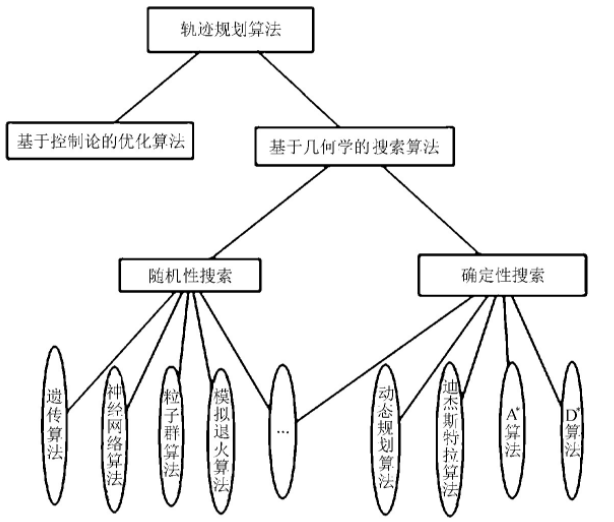图1 常见路径规划算法 文中主要对无人机巡航阶段的航迹规划进行研究，假设无人机在飞行中维持高度与速度不变，那么航迹规划成为一个二维平面的规划问题。在航迹规划算法中,A算法计算简单，容易实现。在改进A算法基础上，提出一种新的、易于理解的改进A算法的无人机航迹规划方法。传统A算法将规划区域栅格化，节点扩展只限于栅格线的交叉点，在栅格线的交叉点与交叉点之间往往存在一定角度的两个运动方向。将存在角度的两段路径无限放大、细化，然后分别用两段上的相应路径规划点作为切点，找到相对应的组成内切圆的圆心，然后作弧，并求出相对应的两切点之间的弧所对应的圆心角，根据下式计算出弧线的长度式中：R———内切圆的半径； α———切点之间弧线对应的圆心角。
二、遗传算法简介
1 引言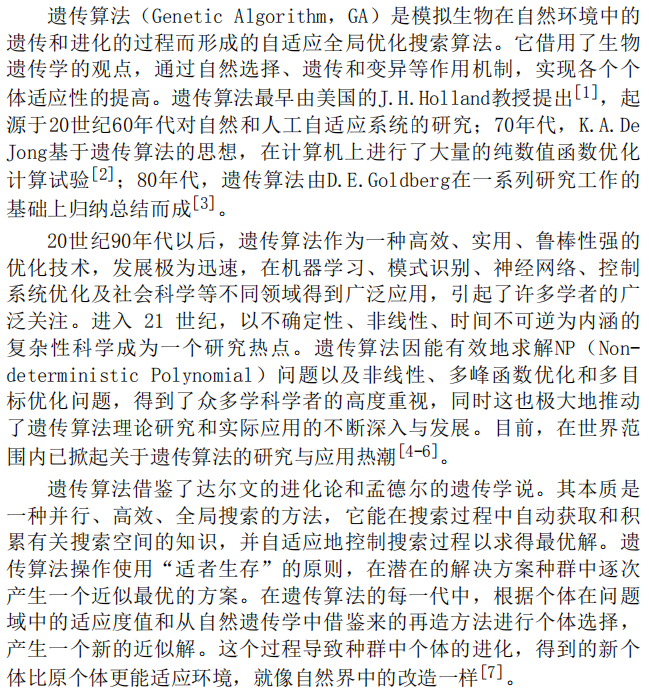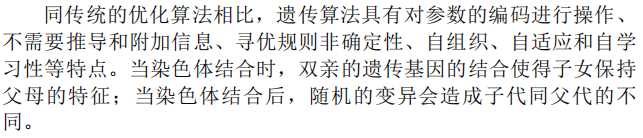2 遗传算法理论 2.1 遗传算法的生物学基础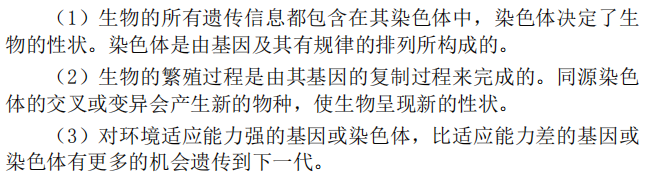2.2 遗传算法的理论基础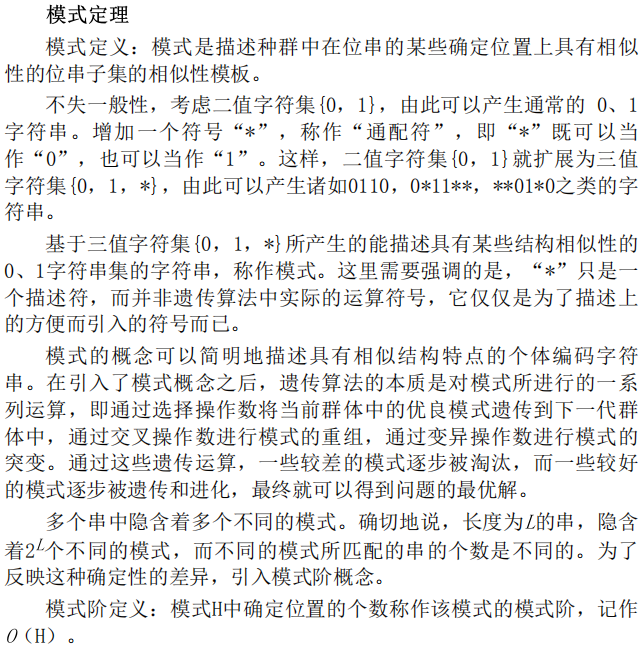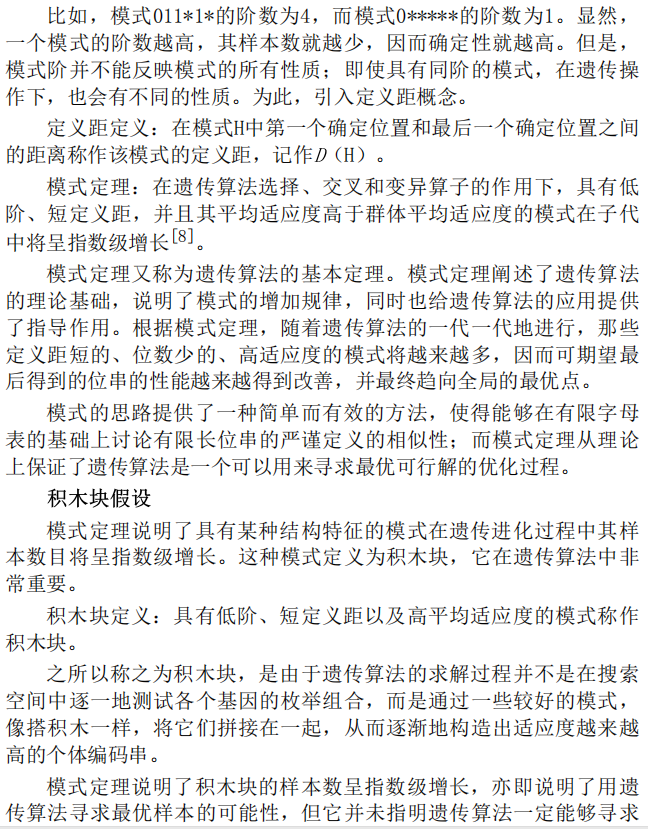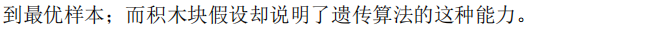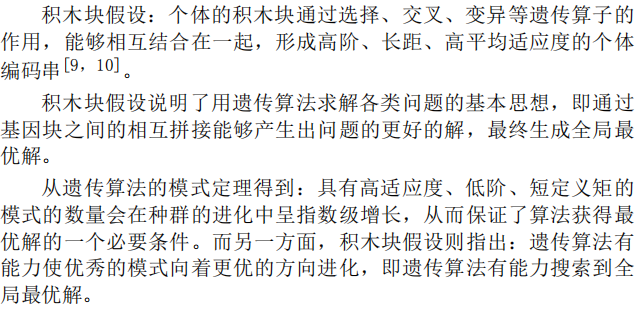2.3 遗传算法的基本概念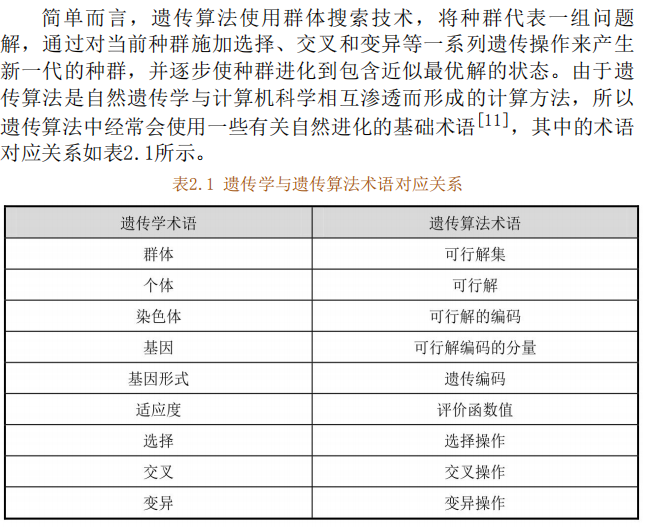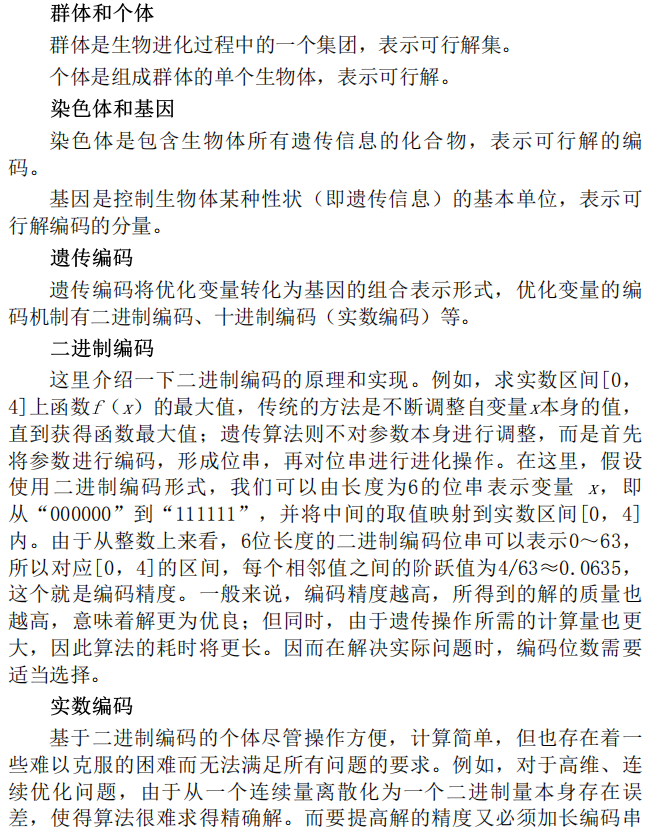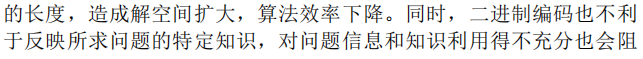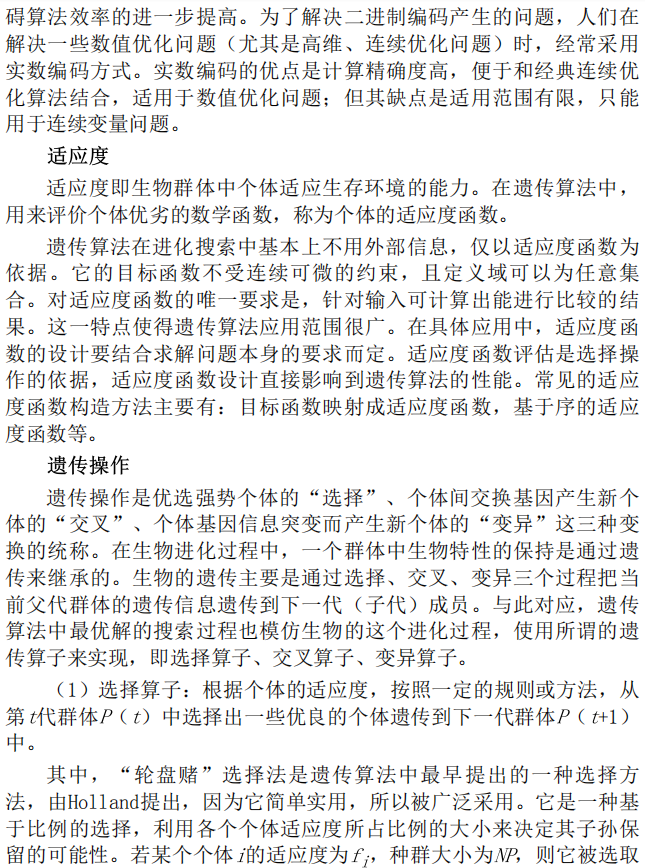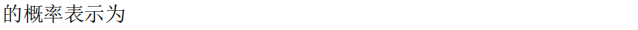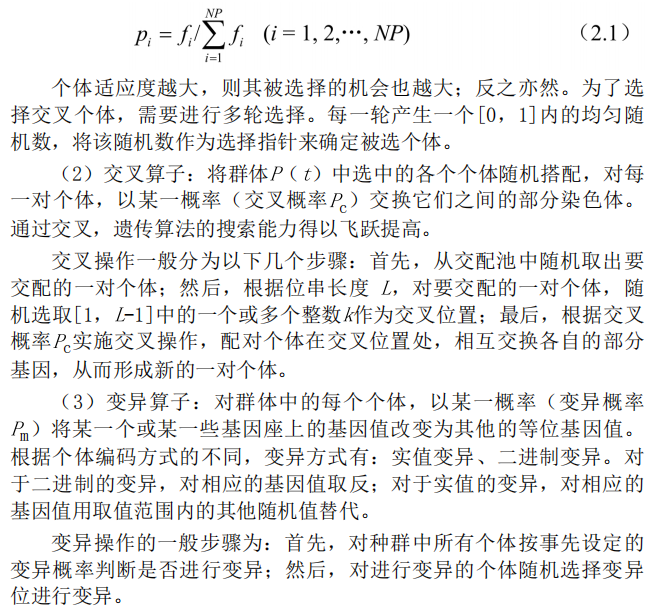2.4 标准的遗传算法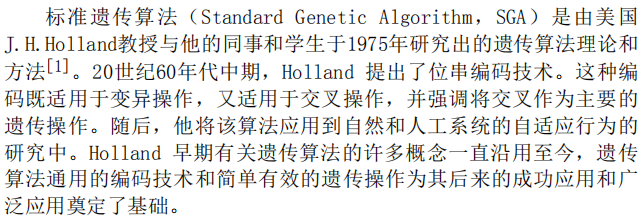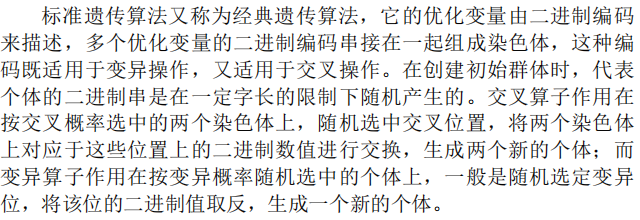2.5 遗传算法的特点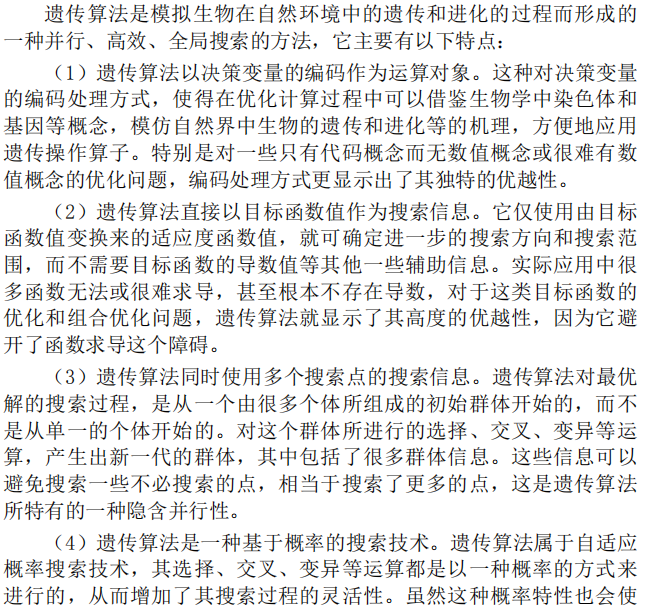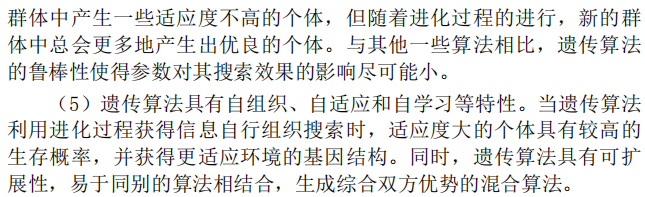2.6 遗传算法的改进方向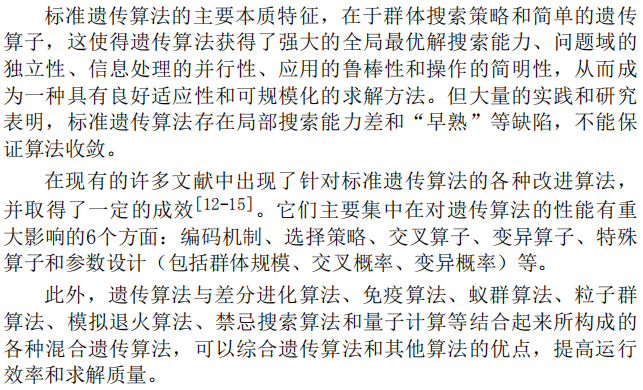3 遗传算法流程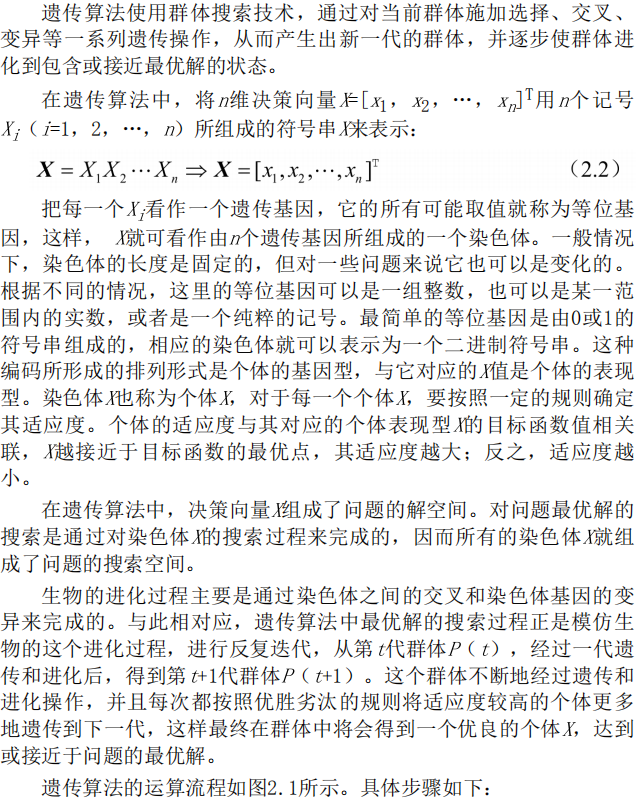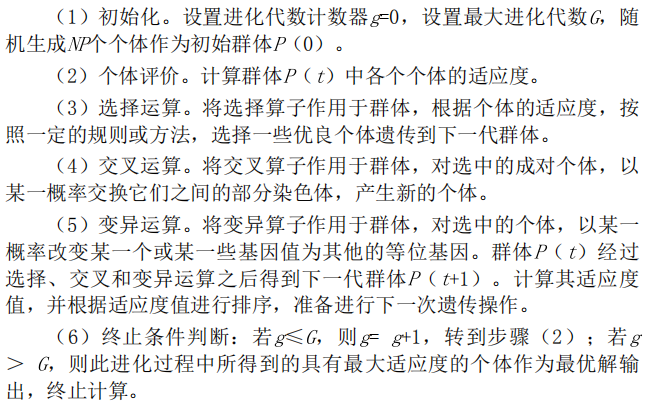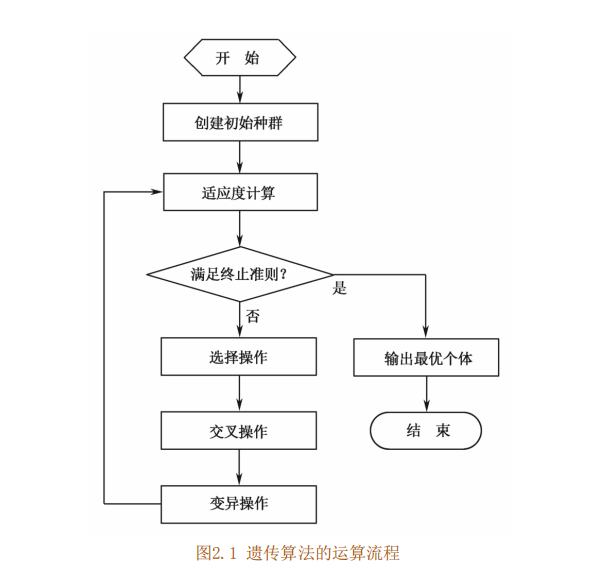4 关键参数说明三、部分源代码
%%%%%%%%%% AUV path planning using GA %%%%%%%%%%
%%%%%Run 'RP_coordinate.m' before running main %%%%

clear all;
close all;
tic;%Runtime timer
%% global variables

Popsize =50;         %Population size, should be an even integer

%Genetic parameters
%MIXRATE = 0.3;
ITERATION = 10000;   %Number of iteration
THRESHOLD = 100;
Pcross = 0.7;       %Crossover rate
Pmutation = 0.3;    %Mutation rate

Fitconst=0;         %Number of generations that fitness values remain constant
%% Genetic algorithm
while(Generation <= ITERATION)
if (Fitconst<=THRESHOLD) %Stop iteration if fitness value is constant in threshold number of genreations
fitness = Fitness(Parentpop,adjacency);       %Calculate fitness of parents
crossover = Crossover(Parentpop,Pcross);      %Crossover
Childpop = Mutation(crossover,Pmutation);     %Mutate and get chindren
combopop=[Parentpop;Childpop];                %Combine parents and chindren
nextpop=Select(combopop,combofitness);        %Select the first half of best to get 2nd gen
Parentpop=nextpop.pop;
if(Generation ==1)
Best_GApath=Parentpop(1,:);
Best_Fitness=combofitness(nextpop.bestplan);
else
New_Best_Fitness=combofitness(nextpop.bestplan);%Evaluate best solution
New_Best_GApath=Parentpop(1,:);

%%%%%%%%Visualize planning process%%%%%%%%
%                     GENERATION=[1:Generation-1];
%                     GAplancoor = [RP(Best_GApath).x;RP(Best_GApath).y; RP(Best_GApath).z].';
%                     figure(1);
%                     for i=1:RPNUM
%                         subplot(2,1,1);     %Plot all rendezvous points
%                         plot3(RP(i).x,RP(i).y,RP(i).z,'o');
%                         text(RP(i).x,RP(i).y, RP(i).z,num2str(i));
%                         hold on;
%                         subplot(2,1,2);
%                         plot(RP(i).x,RP(i).y,'o');
%                         text(RP(i).x,RP(i).y,num2str(i));
%                         hold on;
%                     end
%                     subplot(2,1,1);
%                     plot3(GAplancoor(:,1),GAplancoor(:,2),GAplancoor(:,3),'r-.');
%                     title('3D Path of AUV');
%                     grid on;
%                     hold off;
%                     subplot(2,1,2);
%                     plot(GAplancoor(:,1),GAplancoor(:,2),'r-.');
%                     title('2D Path of AUV');
%                     grid on;
%                     hold off;
%%%%%%%%Visualize planning process%%%%%%%%

else
Fitconst=Fitconst+1;
end
end

Fitnesscurve(Generation)=Best_Fitness;

else

break

end

Generation = Generation +1;

end

toc;
%% plot result plan
GAplancoor = [RP(Best_GApath).x;RP(Best_GApath).y; RP(Best_GApath).z].';
figure(1);
for i=1:RPNUM
subplot(2,1,1);     %Plot all rendezvous points
plot3(RP(i).x,RP(i).y,RP(i).z,'o');
text(RP(i).x,RP(i).y, RP(i).z,num2str(i));
hold on;
subplot(2,1,2);
plot(RP(i).x,RP(i).y,'o');
text(RP(i).x,RP(i).y,num2str(i));
hold on;
end
subplot(2,1,1);
plot3(GAplancoor(:,1),GAplancoor(:,2),GAplancoor(:,3),'r-.');
title('3D Path of AUV');
grid on;
subplot(2,1,2);
plot(GAplancoor(:,1),GAplancoor(:,2),'r-.');
title('2D Path of AUV');
grid on;
%% Plot iteration of fitness
figure(2);
plot(GENERATION,Fitnesscurve,'r.-');
title('Minimum distance in each generation');
xlabel('Generation');
ylabel('Fitness value');
legend('Best Fitness Value');
set(gca, 'Fontname', 'Times New Roman', 'FontSize', 14);
grid on;

% Function for crossover and avoiding conflicts
function crossover = Crossover(pop,Pcross)
crossover=pop;
k=1;
while (k<=(size(crossover,1)-1))
%Russian roulette to decide whether crossover occurs
Pc = unifrnd(0,1);
if(Pc<Pcross)
SS = unidrnd(size(crossover,2)); %Start point of crossover section
SE = unidrnd(size(crossover,2));   %End point of crossover section
while(SS == SE)
SE = unidrnd(size(crossover,2));
end
if(SE<SS) %Order
temp = SE;
SE = SS;
SS=temp;
end
Chrom1=crossover(k,:);          %First chromosome for crossover
Chrom2=crossover(k+1,:);        %Second chromosome for crossover
CS2=Chrom1(SS:SE);        %crossover section 1
CS1=Chrom2(SS:SE);        %crossover section 2
Chrom1(SS:SE)=CS1;        %crossover finished
Chrom2(SS:SE)=CS2;        %crossover finished

%Avoid conflict
LIST=unique(Chrom1); %list all unique numbers
COUNTA=hist(Chrom1,unique(Chrom1)); %Distribute elements on chromosomes to corresponding unique numbers
ISDUP = COUNTA - ones(1,size(COUNTA,2)); %If there is a duplicate number, the result will be non-zero array
DUPElem=LIST(find(ISDUP));          %Find the duplicate elements
ElemPosition=ismember(CS1,DUPElem); %Find the duplicate elements' position
RELATION=zeros(1,size(Chrom1,2));

%Set up relacement relation table
i=1;
while i<=size(CS1,2)
if((ElemPosition(i)==0))
i=i+1;
else
a=CS1(i);
b=CS2(i);
if (~ismember(b,CS1))
RELATION(a)=b;
RELATION(b)=a;
else
while(ismember(b,CS1))
temp=b;
position=find(CS1==temp);
b=CS2(position); %#ok<FNDSB>
end
RELATION(a)=b;
RELATION(b)=a;
end
i=i+1;
end
end
j=1;
%Replacement
while(j<=size(Chrom1,2))
while(j>=SS&&j<=SE)
j=j+1;
end
if(j>size(Chrom1,2))
break
end
if(RELATION(Chrom1(j))==0)
j=j+1;
else
Chrom1(j)=RELATION(Chrom1(j));
j=j+1;
end
end
j=j-1;
while(j~=0)
while(j>=SS&&j<=SE)
j=j-1;
end
if(j==0)
break;
end
if(RELATION(Chrom2(j))==0)
j=j-1;
else
Chrom2(j)=RELATION(Chrom2(j));
j=j-1;
end
end

crossover(k,:)=Chrom1;
crossover(k+1,:)=Chrom2;

end
k=k+2;
end


四、运行结果五、matlab版本及参考文献
1 matlab版本 2014a
2 参考文献  包子阳,余继周,杨杉.智能优化算法及其MATLAB实例（第2版）[M].电子工业出版社，2016. 张岩,吴水根.MATLAB优化算法源代码[M].清华大学出版社，2017. 巫茜,罗金彪,顾晓群,曾青.基于改进PSO的无人机三维航迹规划优化算法[J].兵器装备工程学报. 2021,42(08) 邓叶,姜香菊.基于改进人工势场法的四旋翼无人机航迹规划算法[J].传感器与微系统. 2021,40(07) 马云红,张恒,齐乐融,贺建良.基于改进A*算法的三维无人机路径规划[J].电光与控制. 2019,26(10) 焦阳.基于改进蚁群算法的无人机三维路径规划研究[J].舰船电子工程. 2019,39(03)
展开全文自动驾驶
• 3.用matlab遗传算法，蚁群算法仿真，比较不同算法的优缺点，确定有效的路径规划求解算法。 所以就形成了 遗传算法–>栅格法+TSP问题 蚁群算法–>栅格法+TSP问题 路径优化的问题很常见，本带马工会竭尽所...
这是一个多年以前研究过的课题，现在简单说一下
本课题主要是 1.学习理解机器人路径规划算法，重点研究遗传算法和蚁群算法。 2.对典型的二维场景采用栅格法进行matlab建模。 3.用matlab对遗传算法，蚁群算法仿真，比较不同算法的优缺点，确定有效的路径规划求解算法。
所以就形成了 遗传算法–>栅格法+TSP问题 蚁群算法–>栅格法+TSP问题
路径优化的问题很常见，本带马工会竭尽所能解决小白入坑的问题（手动笑哭）。评论会推送至邮箱，我看见了就会回复。可接私活。 也欢迎各位大佬详细交流，
展开全文蚁群算法
• 遗传算法学习——使用python做路径规划一、引言二、算法伪代码三、算法...  在查阅资料时发现遗传算法路径规划仿真大多采用matlab软件，而python实现的例子几乎没有。再加上本人正好在学习python，就参考matlab代码


遗传算法学习——使用python做路径规划
一、引言二、算法伪代码三、算法流程以及代码实现1、地图创建2、种群初始化小结
3、适者生存之适应度函数小结
4、物竞天择之选择小结
5、遗传学之交叉小结
6、遗传学之变异小结
7、更新种群以及输出结果
四、代码工程文档结束语==问题解决==1、解决种群规模随迭代次数增加而减小的问题

一、引言
机器人控制过程中，路径规划是其中重要的一环。因此本文采用遗传算法对机器人移动时的路径进行优化，最终选取出一条最优路径。采用栅格图法为机器人移动的地图进行建模，并根据遗传算法机理，在python上仿真实现了最优路径的选取。   在查阅资料时发现遗传算法做路径规划仿真大多采用matlab软件，而python实现的例子几乎没有。再加上本人正好在学习python，就参考matlab代码做了python的仿真。 本文重点参考文献链接：基于遗传算法的移动机器人路径规划，在此感谢链接作者。
二、算法伪代码
算法伪代码如下：     step1:建立地图     step2:初始化种群     step3:计算个体的适应度值     step4:选择适应度合适的个体进入下一代     step5:交叉     step6:变异     step7:更新种群，若出现最优路径或达到迭代次数，则转至step8，否则转step3     step8:输出最优的个体作为最优解   本文会按照算法的伪代码一步一步讲解。
三、算法流程以及代码实现
1、地图创建
本文采取栅格图法创建地图空间，便于确定直角坐标系。   如图1所示，图中共有25个栅格，每个栅格表示一段路径，其中黑色方块表示的是障碍物，白色方块表示的是自由栅格，即可行走。   以图中为例，在构建栅格地图时，首先以地图左下角第一个栅格为坐标原点建立直角坐标系。因此每一个栅格可以用（x,y）的坐标形式表示，比如左下角第一个栅格可以表示为（1,1）。并且从左下角开始每一个栅格进行编号N，编号从0开始，设定编号0为起点，24为终点。说明下，这种编号方式就是遗传算法中的编码操作，会使相对复杂的问题结构，变得简单。比如现在以坐标形式表示一条从（1，1）到（5，5）的路径：（1，1）->（2，2）->（3，2）->（4，2）->（4，3）->（5，4）->（5，5）。这种方法在编程中需要使用二维数组来存放这么一条路径，但通过编码之后，这样的路径可以简化为：（0，6，7，8，13，19，24），只需要一个向量便可以表示。其中坐标和编码之间的转换关系为：

x

=

N

/

c

o

l

+

1

x = N/col+1

y

=

N

%

c

o

l

+

1

y = N\%col+1

其中col为栅格图的列数，N为编码，x,y分别为横纵坐标,也可读作x行y列（需要注意，行数从下往上数，列数从左往右数）。以上方法以一种通用的方法，本案例代码中由于使用python编程，构建地图用的是列表的方式，因此行数从上往下，列数从左往右，并且由于列表的索引从0开始，因此坐标原点也是用的（0，0）如下图所示，光标选中的左上角元素坐标为（0，0），编码为0，编码从左往右一次是0，1，2…直到右下角的99。python实现画栅格地图的代码如下：
import random
class Map():
'''
:param:地图类
:param:使用时需要传入行列两个参数 再实例化
'''

def __init__(self,row,col):
'''
:param:row::行
:param:col::列
'''
self.data = []
self.row = row
self.col = col
def map_init(self):
self.data = [[0 for i in range(self.col)] for j in range(self.row)]
# for i in range(self.row):
#     for j in range(self.col):
#         print(self.data[i][j],end=' ')
#     print('')
def map_Obstacle(self,num):
'''
:param:num:地图障碍物数量
:return：返回包含障碍物的地图数据
'''
self.num = num
for i in range(self.num):#生成小于等于num个障碍
self.data[random.randint(0,self.row-1)][random.randint(0,self.col-1)] = 1
if self.data == 1:        #判断顶点位置是否是障碍  若是 修改成可通行
self.data = 0

if self.data[self.row-1] == 1:
self.data[self.row-1] = 0

if self.data[self.col-1] == 1:
self.data[self.col - 1] = 0

if self.data[self.row-1][self.col - 1] == 1:
self.data[self.row - 1][self.col - 1] = 0
for i in range(self.row):       #显示出来
for j in range(self.col):
print(self.data[i][j],end=' ')
print('')
return self.data

使用时代码如下：
map = Map(10,10)#10行 10列
map.map_init()#生成初始的10行10列 的0矩阵
arr = map.map_Obstacle(20)#这里传入的参数是在10*10 的0矩阵上随机生成20个障碍物，因为是随机生成，实际的障碍物数量<=20 返回带有障碍的数据给arr

到这里，已经生成了一个带有障碍的栅格地图，现在进行step2
2、种群初始化
种群的概念是个体的集合。在这之前需说明遗传算法是从问题解的编码组开始，而非单个解开始搜索。即种群的每一个个体都是当前问题的一个可行解，使用遗传算法的过程，是从这些可行解（个体）中找出最优解（适应度最强的个体）。对于本文来讲，种群的初始化就是找到若干组可以从起点到达终点的路径，每一组路径就是一个个体，种群的大小就是个体的数量。遗传算法求解最优路径过程中，种群的初始化是一个难点，也是最难的一步，既要保证路径的可行性，还要保证路径的连续性（即不能跳跃行走）。在遗传算法路径规划中，种群的初始化过程是最重要的，也是最难的。下面开始介绍本文用到的方法。   本文实现种群初始化分为两步，第一步为找到一条间断的路径，即从栅格图的每一行中随机取出一个不是障碍物的元素。比如在图1中取（0，6，10，16，24），在每一行中都取了一个元素，并且这些元素都是自由栅格。第二步为将间断的路径连续化，在这步中，需要先从路径的第一个栅格判断此条路径与之相邻的下一个栅格是否为连续，判断公式为：

D

=

M

a

x

{

a

b

s

(

x

1

−

x

2

)

,

a

b

s

(

y

1

−

y

2

)

}

D = Max\{abs(x1-x2),abs(y1-y2)\}

若D=1，则说明路径连续，反之不连续。对于不连续的两个坐标，采用中点法计算两个栅格的中点栅格，公式如下：其中（X_new，Y_new）是新插入栅格的坐标。  1.若新栅格为障碍物栅格：则以上下左右(这个顺序可以任意，也可以改为8个方向搜索)顺序取新栅格的相邻栅格，并判断此栅格是否已经在路径中。   a).若此栅格为无障碍栅格且不在路径中则插入路径中；   b).若遍历上下左右四个栅格后没有满足条件的栅格则删除这条路径；  2.若新栅格为无障碍物栅格，则插入两个到不连续栅格中间。  3.继续判断新插入的栅格和新插入的栅格的前一个栅格是否连续，若不连续则循环以上步骤，直到两个栅格连续。当两个栅格连续后取下一个栅格，循环以上步骤，直到整条路径连续。  4.按照以上步骤生成了一条初始路径之后，会出现走回头路的现象，比如按照图1生成了以下一条路径：（0，6，1，2，6，7，8，13，19，24）可以看出，6号这个节点一共走了两次，这样的话这条路径就会有冗余部分，也就是我认为的走了回头路。这种情况下，为了使算法加快收敛速度，就要保证初始种群比较优良，也就是初始的路径长度就短，那么就有必要滤除这段重复的路段，这里专门设计了一个滤除一段路径中两个相同元素之间内容的算法。代码在我的另一篇博文里面，这里附上链接：python实现将列表中重复元素之间的内容全部滤除   这里初始化种群的步骤十分繁琐，因此直接上初始化代码，代码上有详细注释，代码如下：
#step2 初始化种群 也是最难的一步
class Population():
def __init__(self,row,col,num,NP):
self.row = row
self.col = col
self.num = num
self.NP = NP
self.p_start = 0  # 起始点序号  10进制编码
self.p_end = self.row * self.col - 1  # 终点序号 10进制编码
self.xs = (self.p_start) // (self.col)  # 行
self.ys = (self.p_start) % (self.col)  # 列
self.xe = (self.p_end) // (self.col)  # 终点所在的行
self.ye = (self.p_end) % (self.col)
self.map_start = Map(self.row, self.col)  # Map的实例化 主函数中可以不再初始化地图
self.map_start.map_init()  # map初始化 生成0矩阵
self.map = self.map_start.map_Obstacle(self.num)  # 生成带障碍的map

self.can = []  # 这个列表存放整个地图中不是障碍物的点的坐标 按行搜索
self.popu = [[0 for i in range(self.col)]
for j in range(self.NP)]  # 种群的列表，包含NP个间断可行解，即从起点到终点的路径
# self.popu = []#存放可行解  可行路径
self.end_popu = []	#存放最终的连续NP条路径

def Population_Init(self):
'''
:return:返回NP条连续可行解  即初始路径
'''
for i in range(self.NP):       #添加NP条路径
j = 0
for xk in range(0,self.row):  #多少行
self.can = []             #清空can列表   用来缓存当前行的可行点
for yk in range(0,self.col):   #从起点0 开始的列开始搜寻到终点的列  也就是此行中的自由点
num = (yk) + (xk) * self.col
if self.map_start.data[xk][yk] == 0:
self.can.append(num)
# print(self.can,'自由点\n')
length = len(self.can)     #此行的长度  随机生成指针取出坐标
# print(length)
self.popu[i][j] = (self.can[random.randint(0,length-1)])
j += 1#j从1 开始  是因为 0 的元素时起点编号
self.popu[i] = self.p_start  # 每一行的第一个元素设置为起点序号
self.popu[i][-1] = self.p_end

temp = self.Generate_Continuous_Path(self.popu[i])#将返回的一条路经 添加进end中
if temp != []:      #将返回的空列表路径滤除
temp = fiter.fiter(temp)    #滤除重复节点路径
self.end_popu.append(temp)
# print(self.end_popu, end='\n')
# print('测试1',self.popu,end='\n')
return self.end_popu
# @staticmethod
def Generate_Continuous_Path(self,old_popu):#生成连续的路径个体
'''
:param old_popu: 未进行连续化的一条路径
:return:        返回已经连续化的一条路径
'''
self.new_popu = old_popu    #传进来的参数就是一行的数组  一条路径
self.flag = 0
self.lengh = len(self.new_popu)  #先将第一条路经的长度取出来
i = 0
# print("lengh =",self.lengh )
while i!= self.lengh-1:       #i 不等于 当前行的长度减去1  从0计数  这里有问题 待修改
x_now = (self.new_popu[i]) // (self.col)  # 行 解码  算出此条路经的第i个元素的直角坐标
y_now = (self.new_popu[i]) % (self.col)  # 列
x_next =  (self.new_popu[i+1]) // (self.col) #计算此条路经中下一个点的坐标
y_next =  (self.new_popu[i+1]) % (self.col)
#最大迭代次数
max_iteration = 0

#判断下一个点与当前点的坐标是否连续 等于1 为连续
while max(abs(x_next - x_now), abs(y_next - y_now)) != 1:
x_insert = math.ceil((x_next + x_now) // 2)      #行
y_insert = math.ceil((y_next + y_now) // 2) #ceil向上取整数     #列
# print("x_insert = ",x_insert,"\ty_insert = ",y_insert)
flag1 = 0

if self.map_start.data[x_insert][y_insert] == 0:  #插入的这个坐标为0 可走
num_insert = (y_insert) + (x_insert) * self.col #计算出插入坐标的编码
self.new_popu.insert(i+1,num_insert)
# print(self.new_popu)
# print(num_insert)
else:#插入的栅格为障碍  判断插入的栅格上下左右是否为障碍，以及是否在路径中，若不是障碍且不在路径中，就插入
#判断下方
if (x_insert + 1 < self.row)and flag1 == 0:       #保证坐标是在地图上的
if ((self.map_start.data[x_insert+1][y_insert] == 0)#下方不是障碍物
and (((y_insert) + (x_insert+1) * self.col) not in self.new_popu)):#编号不在已知路径中
num_insert = (y_insert) + (x_insert+1) * self.col  #计算下方的编号
self.new_popu.insert(i + 1, num_insert) #插入编号
flag1 = 1       #设置标志位 避免下面重复插入

# print('下方插入',num_insert)
#判断右方
if (y_insert + 1 < self.col)and flag1 == 0:  # 保证坐标是在地图上的 并且前面没有插入
if ((self.map_start.data[x_insert][y_insert+1] == 0)#右方不是障碍物
and (((y_insert+1) + (x_insert) * self.col) not in self.new_popu)):#编号不在已知路径中
num_insert = (y_insert+1) + (x_insert) * self.col  #计算右方的编号
self.new_popu.insert(i + 1, num_insert) #插入编号
flag1 = 1  # 设置标志位 避免下面重复插入
# print('右方插入',num_insert)
#判断上方
if (x_insert - 1 > 0) and flag1 == 0:  # 保证坐标是在地图上的
if ((self.map_start.data[x_insert-1][y_insert] == 0)#右方不是障碍物
and (((y_insert) + (x_insert-1) * self.col) not in self.new_popu)):#编号不在已知路径中
num_insert = (y_insert) + (x_insert-1) * self.col  #计算右方的编号
self.new_popu.insert(i + 1, num_insert) #插入编号
flag1 = 1  # 设置标志位 避免下面重复插入
# print('上方插入',num_insert)
#判断左方
if (y_insert - 1 > 0)and flag1 == 0:  # 保证坐标是在地图上的
if ((self.map_start.data[x_insert][y_insert-1] == 0)#右方不是障碍物
and (((y_insert-1) + (x_insert) * self.col) not in self.new_popu)):#编号不在已知路径中
num_insert = (y_insert-1) + (x_insert) * self.col  #计算右方的编号
self.new_popu.insert(i + 1, num_insert) #插入编号
flag1 = 1  # 设置标志位 避免下面重复插入
# print('左方插入',num_insert)
if flag1 == 0:  #如果前面没有插入新点  说明这条路径不对 删除
self.new_popu = []
break
x_next = num_insert//self.col
y_next = num_insert%self.col
# x_next = x_insert
# y_next = y_insert
max_iteration += 1#迭代次数+1
if max_iteration > 20:
self.new_popu = []  #超出迭代次数 说明此条路经可能无法进行连续   删除路径
break
if self.new_popu == []:
break
self.lengh = len(self.new_popu)
i = i+1
# print(self.new_popu,'连续')
return  self.new_popu#返回的是一条路径

这里需要注意的是，在种群初始化对象的__init__方法中已经初始化了地图数据，因此主函数只需要对种群初始化对象实例化就行，使用方法如下：
population = Population(10,10,20,100)   #实例化对象 并生成初始带随机障碍的地图
popu = population.Population_Init()  #种群初始化  得到np条初始可行路径

小结
种群初始化是最难的一步，因为需要判断的地方非常多，比如连续化路径时，还要判断是否超出了地图边界，因为地图是列表数据，一旦超出索引，程序就会报错。还有许多需要注意的地方，这些在程序中都有注释，此外前文提到如果插入的点是障碍物，那么就在其上下左右四个方向搜寻自由点，其实也可以改为在其周围8个方向搜寻自由点，毕竟对角线的方向也是可以走的。   然而出现的问题还是有的，使用中点插值法使路径连续化会产生一些错误，有的时候会穿过障碍行驶。针对这个问题，在下一步计算适应度函数的过程中，使用了惩罚函数来惩罚这些穿越障碍物的路径，但这个问题是这种方法本身的局限，靠惩罚函数是不能彻底解决的。因此在一些文献中，初始化种群还会采用RRT随机树的方法，这个在之后会改进。   到这里初始化种群结束，进行下一步，计算适应度函数。
3、适者生存之适应度函数
这里就进入到了达尔文进化论的范畴了，其实并没有那么玄乎．适应度函数就是衡量一个问题的解是否是最优的标准，比如一个适应度函数：

f

(

x

)

=

x

2

+

1

f(x) = x^2+1

要求f(x)的值取得最小时,x的值是最优的,那么f(x)就是适应度函数的值,x取得每一个值都是适应度函数的解,问题就是这个解是不是最优的。归根到底，遗传算法其实就是解决这样一个问题的方法。   而本文选取的适应度函数标准为使得路径最短。代码中给定相邻的栅格图为1个单位长度，则对角的长度为1.4（根号2）。其实除了路径最短这一标准外，还有路径平滑度，机器人能耗等标准，由于能力有限，故本文只采用路径最短作为标准。   根据以上的解释，求适应度函数值就可以理解为求一条路径从起点到终点的距离。代码如下：
#step3 计算适应度函数
def calvalue(popu,col):
'''
:param popu: 传入种群信息
:param col: 这个参数是地图的列数 ，因为要解码用到
:return: 返回的是每一条路径长度组成的列表
'''
hang = len(popu)#计算行
value = [] #存放计算出来的路径长度值
for i in range(hang):#从第0行开始 到最后一行
value.append(0)
single_popu = popu[i] #把当前行的元素赋给 single_popu 之后操作
single_lengh = len(single_popu)#将当前行的长度拿出来
for j in range(single_lengh-1):#从第一个元素计算到倒数第一个元素
x_now = (single_popu[j]) // (col)  # 行 解码  算出此条路经的第i个元素的直角坐标
y_now = (single_popu[j]) % (col)  # 列
x_next = (single_popu[j + 1]) // (col)  # 计算此条路经中下一个点的坐标
y_next = (single_popu[j + 1]) % (col)
if abs(x_now - x_next) + abs(y_now - y_next) == 1:#路径上下左右连续 不是对角的 则路径长度为1
value[i] = value[i]+1
elif max(abs(x_now - x_next),abs(y_now - y_next))>=2:#惩罚函数 若跳跃或者穿过障碍
value[i] = value[i] + 100
else:
value[i] = value[i]+1.4  #对角长度为根号2  即1.4
return value

小结
在计算适应度值的时候，传入的种群路径有的其实并不连续，因为跨过了障碍，因此在函数中用以下代码进行惩罚：
elif max(abs(x_now - x_next),abs(y_now - y_next))>=2:#惩罚函数 若跳跃或者穿过障碍
value[i] = value[i] + 100

就是将路径长度直接加长，这样的话在下一步＂选择＂操作中就大概率的会将这样的路径过滤掉，但不能保证全部过滤。
4、物竞天择之选择
这里就是根据适应度值进行选择比较优良的个体存活到下一代，也就是物竞天择。本文中采取了两种方法进行选择优良个体进入下一代。第一种方法是参考文献中所采用的轮盘赌方法；第二种方法是将适应度值从小到大排序，假设种群中强者的概率是50%，即0.5，并且认为强者都能存活（这里强者的概率其实就是对强者筛选过了），即都能进入下一代。那么剩下的50%就是弱者，而对于弱者，也有一个弱者存活概率比如0.3，之后采取随机存活的方式，产生一个0-1之间的随机小数,若0.3大于这个小数 ,那么认为可以存活，否则直接舍弃。存活下来的强者和弱者构成了一个新的种群，进行下一步的交叉操作。首先来看参考文献中的轮盘赌方法进行筛选下一代，代码如下：
#step4 选择
def selection(pop,value):
'''
:param pop:种群
:param value:适应度值列表
:return:返回新的种群
'''

###原来的方法会丢失种群数量
now_value=[]#做倒数后的适应度值列表
P_value = []  #存放适应度值占总比概率的列表
random_deci = []#存放随机的小数
new_popu = []       #选择后的种群
sum_value = 0   #存放适应度的总和  计算轮盘赌的概率
lengh = len(pop)#首先计算种群有多少行，也就是有多少初始路径
for i in range(lengh):
new_popu.append([]) #原始种群有多少个个体  新的种群就有多少
for i in value:     #由于适应度值是距离  将需要的就是距离最短的  因此求倒数来排序
now_value.append(1/i)  #将适应度取倒数   倒数越小适应度越小，路径越长  就可以按概率抛弃
sum_value += (1/i)
for i in now_value:#将每一个适应度值取出来  做比例  得出每个适应度值的占比概率 存到列表中
P_value.append(i/sum_value)
P_value = np.cumsum(P_value)#累加和 并排序 从小到大
for i in range(lengh):
random_deci.append(random.random())#产生 i个随即小数 存到列表中
random_deci = sorted(random_deci)#从小到大排序
fitin = 0
newin = 0
while(newin<lengh): #遍历每一行
if random_deci[newin] < P_value[fitin]:
new_popu[newin] = pop[fitin]#这里的代码有点难理解
newin += 1
else:
fitin += 1
return new_popu

再看第二种方法，代码如下：
#step4 选择
def selection(pop,value):
'''
:param pop:种群
:param value:适应度值列表
:return:返回新的种群
'''
####原来的方法会丢失种群数量
retain_rate = 0.6#适应度最优的保留几率
random_rate = 0.3#适应度差的保留几率
dict_my = dict(zip(value, pop))     #将路径距离和路径打包字典

new_popu = []
sort_dis = sorted(dict_my)   #将字典按照键排序  也就是按照距离短-长排序
retain_lengh = int(len(pop)*retain_rate)      #适应度强的按照存活概率 保留下
temp = sort_dis[:retain_lengh]      #缓存下保留的距离，待会将距离对应的字典值取出来
# print(temp,'优秀保留')
for i in sort_dis[retain_lengh:]:   #距离长的按照随机概率保留
if random.random() < random_rate:
temp.append(i)
#temp现在存放的就是进入下一代的种群信息，他是距离信息，下一步通过字典将种群提取出来
for i in temp:
new_popu.append(dict_my[i]) #至此种群选取完
return new_popu

小结
这两种方法都能够选取下一代的种群，但都会出现一个问题：在选择的过程中，种群的规模会慢慢变小，有时侯不等迭代结束，种群就所剩无几（即可行路径没剩几条了）虽然能够选出比较优的路径解，但总感觉哪里不对以及路径不是最优的，因此后续还要再查资料，解决这个问题。
5、遗传学之交叉
交叉的方法有许多种，由于这里采用十进制编码方式，因此单点交叉方法比较简单实用。   代码中的思想为：给定一个交叉概率pc，然后再产生0-1之间的一个随机数，并和交叉概率pc比较，若pc大于这个随机小数，则进行交叉操作。   交叉操作具体为：由于路径的不同而导致个体长度的不同,因此,选择相邻的两个个体,其交叉点的位置不一定相同,这里选取相同编码处进行交叉(起点和终点除外),以保证路径的连续性。具体的交叉操作是找出两条路径中所有相同的点，然后随机选择其中的一个点，将之后的路径进行交叉操作。比如一条连续路径为（0，6，7，8，13，18，23，24），另一条连续路径为（0，1，6，7，8，13，19，24），重复的序号（6，7，8，13），则随机选取一个序号，如13，将13后面的序号交叉，即可得到两条新的路径（0，6，7，8，13，19，24）和（0，1，6，7，8，13，18，23，24），其中（0，6，7，8，13，19，24）其实是肉眼看到的最优的路径，这就是交叉的操作。 代码如下：
#step5 交叉   拟采用单点交叉
def cross(parents,pc):
'''
:param parents: 交叉的父类
:param pc:   交叉概率
:return:
'''
children = []  #首先创建一个子代 空列表 存放交叉后的种群
single_popu_index_list = []#存放重复内容的指针
lenparents = len(parents)  #先提取出父代的个数  因为要配对 奇数个的话会剩余一个
parity = lenparents % 2 #计算出长度奇偶性  parity= 1 说明是奇数个  则需要把最后一条个体直接加上 不交叉
for i in range(0,lenparents-1,2):       #每次取出两条个体 如果是奇数个则长度要减去 一  range函数不会取最后一个
single_now_popu = parents[i]   #取出当前选中的两个父代中的第一个
single_next_popu = parents[i+1]#取出当前选中的两个父代中的第二个
children.append([]) #子代添加两行  稍后存取新的种群
children.append([])
index_content = list(set(single_now_popu).intersection(set(single_next_popu))) #第一条路经与第二条路经重复的内容
num_rep = len(index_content)          #重复内容的个数
if random.random() < pc and num_rep>=3:
content = index_content[random.randint(0,num_rep-1)]   #随机选取一个重复的内容
now_index = single_now_popu.index(content)  #重复内容在第一个父代中的索引
next_index = single_next_popu.index(content)#重复内容在第二个父代中的索引
children[i] = single_now_popu[0:now_index + 1] + single_next_popu[next_index + 1:]
children[i+1] = single_next_popu[0:next_index + 1] + single_now_popu[now_index + 1:]
else:
children[i] = parents[i]
children[i+1] = parents[i+1]
if parity == 1:     #如果是个奇数  为了保证种群规模不变 需要加上最后一条
children.append([]) #子代在添加一行
children[-1] = parents[-1] #直接遗传给下一代
return children

这里如果pc概率小于随机小数，不进行交叉操作的话，就把原来的路径信息直接赋给下一代，因为要保证交叉操作不会减小种群规模。还有一点要注意，如果父代种群有奇数条路径，可以看到，代码中是每次选择相邻的两条路径进行交叉，只能交叉偶数个父代，那么最后会剩余一个父代没法交叉，这里就是直接遗传给下一代了。
    if parity == 1:     #如果是个奇数  为了保证种群规模不变 需要加上最后一条
children.append([]) #子代在添加一行
children[-1] = parents[-1] #直接遗传给下一代

小结
交叉操作是肯定不会减小种群的规模的，并且还会使种群多样化，在写这篇博客时，忽然想到，如果交叉时选择的父代是随机选择，指定交叉次数，会不会能使种群的规模达到我所指定的规模？还有一个之前就想到的问题，如果相同的元素除了起始点和交叉点之外大于等于2个，那么父代交叉完一次后，子代可能还会有重复的元素，子代是否能再次交叉一次？直至两个子代没有相同元素。这些操作能够使种群更加多样化，并且有可能解决种群规模在选择操作时减小的问题。这里记下，之后检验。
6、遗传学之变异
遗传学基因突变是指基因中的某一段发生变化，在此路径规划中的变异就是指一条路径的某一段发生了变化，在代码中的思想为：给定一个变异概率pm，然后产生0-1之间的一个随机数，并和变异概率pm比较，若pm大于随机小数则进行变异操作。   变异方法是随机选取路径中除起点和终点以外的两个栅格，去除这两个栅格之间的路径，然后以这两个栅格为相邻点，使用初始化种群中的路径连续化步骤将这两个点进行连续操作。此时有可能无法产生连续的路径，则需要重新选择两个栅格执行以上操作，直到完成变异操作。这种重新生成的连续路径就是变异之后的连续路径。 变异代码如下：
#step6 变异
def mutation(children,pm):
'''
:param children: 子代种群
:param pm: 变异概率
:return: 返回变异后的新种群
'''

row = len(children)   #子代有多少行   即多少条路经
new_popu = []
for i in range(row):#提取出来每一行

single_popu = children[i]
if random.random()<pm:#小于变异概率   就变异
col = len(single_popu)#每一行的长度 即列数  也就是这条路径有多少节点
first = random.randint(1,col-2) #随机选取两个指针
second = random.randint(1,col-2)
if first != second :    #判断两个指针是否相同  不相同的话把两个指针中间的部分删除 在进行连续化
#判断一下指针大小  便于切片
if(first<second):
single_popu = single_popu[0:first]+single_popu[second+1:]
else :
single_popu = single_popu[0:second] + single_popu[first+1:]
temp = population.Generate_Continuous_Path(single_popu)#连续化
if temp!= []:
new_popu.append(temp)
else:       #不变异的话就将原来的个体直接赋值
new_popu.append(single_popu)
return new_popu

小结
额。。。这个代码贴上来之后，我好像发现了种群规模减小的原因了。原因就是：此时有可能无法产生连续的路径，则需要重新选择两个栅格执行以上操作，直到完成变异操作。这句话我在程序中忽略了，对于无法生成路径的变异个体，我直接舍弃了，所以会导致变异丢失种群数量。在程序中应该判断如下：
if temp == []:#路径为空，说明没有生成连续路径
'''
这段里面应该在进行循环变异知道变异成功
'''
pass

7、更新种群以及输出结果
至此，选择、交叉、变异全部完成，种群更新完毕。若没有出现最优路径或者超出迭代次数，则继续进行之前的操作。若是出现最优路径或迭代完毕则从种群中找到适应度值最小的个体进行输出，并将栅格地图中的编码用’*‘代替。画出清晰的路径图。 这段代码在主函数中呈现：
if __name__ == '__main__':
print('原始地图')
population = Population(10,10,20,100)   #实例化对象 并生成初始带随机障碍的地图

popu = population.Population_Init()  #种群初始化  得到np条初始可行路径
# print(popu,'连续路径')#打印出np条可行路径

for i in range(200):    #迭代200代
lastpopu = popu #上次的种群先保存起来
value = calvalue(popu,population.col) #计算适应度值
# print(value,'适应度值')#打印出np条路径的适应度值

new = selection(popu,value)#选择 按适应度返回新的种群
# print(new,'新种群')
# value = calvalue(new,population.col) #计算适应度值
# print(value,'新种群适应度值')#打印出np条路径的适应度值
child = cross(new,0.8)  #交叉  产生子代
# print(child,'交叉子代')
# value = calvalue(child,population.col) #计算适应度值
# print(value,'子代适应度值')#打印出np条路径的适应度值
popu = mutation(child,0.8)#变异 产生子代 并更新原始种群

if popu == []:  #如果本次的种群成空了  则把上次的种群信息拿出来，并迭代结束
popu = lastpopu
break
# print('第',i,'次迭代后的种群为：',popu)
if popu == []:  #迭代完成后先判断 若迭代完成了 但是种群路径还是空 说明可能是没有路径
print('无路径')
else:
value = calvalue(popu,population.col) #计算适应度值
minnum = value
for i in range(len(value)):#找出最小的适应度函数值
if value[i] < minnum:#小于最小适应度  则替代
minnum = value[i]
popu = popu[value.index(minnum)]#取出最小的适应度值的个体

for i in popu:
x = (i) // (population.map_start.col)  # 行 解码  算出此条路经的第i个元素的直角坐标
y = (i) % (population.map_start.col)  # 列
population.map_start.data[x][y] = '*'   #将路径用*表示
print('\n规划地图')
for i in range(population.map_start.row):   #显示路径
for j in range(population.map_start.col):
print(population.map_start.data[i][j],end=' ')
print('')
print('最短路径值为：',minnum)
print('最短路径为：',popu)

结果如下图，上面的数组为生成的带障碍的原始地图，下面的为规划好路径的地图，最后输出最优路径的路径长度以及路径编码号。四、代码工程文档
理论再多，不如直接上可以用的代码，下面的代码为整体的工程代码，直接可Ctrl+C->Ctrl+V运行:
# -*- coding: UTF-8 -*-
'''*******************************
@ 开发人员：Mr.Zs
@ 开发时间：2020/5/18 19:22
@ 开发环境：PyCharm
@ 项目名称：算法类总工程->遗传算法路径规划V1.0.py
******************************'''
import random   #生成随机整数
import numpy as np #生成随机小数
import math #用于计算除法 取整等运算
print(r'''
遗传算法是对参数集合的编码而非针对参数本身开始进化
遗传算法是从问题解的编码组开始，而非单个解开始搜索

step1:建立地图
step2:初始化种群（随机生成若干条从起点能够到达终点的路径(可行解)，每一条可行路径为一个个体）
step3:计算个体的适应度值
step4:选择适应度合适的个体进入下一代
step5:交叉
step6:变异
step7:更新种群，若没有出现最优个体，则转至step3
step8:输出最优的个体作为最优解
''')

#这个对象专门滤除路径中的回头路
class Fiter:
def __init__(self):
self.b = 1  # 标志位

def function(self, a):  # 定义一个函数
for i in a:  # 遍历列表中的内容
a = a[a.index(i) + 1:]  # 把当前内容索引的后面的内容剪切下来  因为前面的已经比对过了
if i in a:  # 如果当前内容与后面有重复
return i, 1  # 返回当前重复的内容 以及标志位1
else:  # 没有重复就不用管  继续for循环
pass
return 0, 0  # 全部遍历完  没有重复的就返回0 这里返回两个0 是因为返回的数量要保持一致

def fiter(self, a):
while (self.b == 1):  # 标志位一直是 1  则说明有重复的内容
(i, self.b) = self.function(a)  # 此时接受函数接收 返回值 i是重复的内容 b是标志位
c = [j for j, x in enumerate(a) if x == i]  # 将重复内容的索引全部添加进c列表中
a = a[0:c] + a[c[-1]:]  # a列表切片在重组
return a
fiter = Fiter()#实例化对象
#将地图上的点抽象话，可以用x表示横坐标 y表示纵坐标
class Point:
def __init__(self, x, y):
self.x = x
self.y = y

def __eq__(self, other): #函数重载  #判断两个坐标  的  值 是否一样
if((self.x == other.x )and (self.y == other.y)):
return  True
else:
return False
def __ne__(self, other):
pass

#step1
class Map():
'''
:param:地图类
:param:使用时需要传入行列两个参数 再实例化
'''

def __init__(self,row,col):
'''
:param:row::行
:param:col::列
'''
self.start_point = Point(0,0)
self.end_point = Point(row-1,col-1)
self.data = []
self.row = row
self.col = col
def map_init(self):
'''
:param:创建栅格地图row行*col列 的矩阵
'''
self.data = [[0 for i in range(self.col)] for j in range(self.row)]
# for i in range(self.row):
#     for j in range(self.col):
#         print(self.data[i][j],end=' ')
#     print('')
def map_Obstacle(self,num):
'''
:param:num:地图障碍物数量
:return：返回包含障碍物的地图数据
'''
self.num = num
for i in range(self.num):#生成小于等于num个障碍
self.data[random.randint(0,self.row-1)][random.randint(0,self.col-1)] = 1
if self.data == 1:        #判断顶点位置是否是障碍  若是 修改成可通行
self.data = 0

if self.data[self.row-1] == 1:
self.data[self.row-1] = 0

if self.data[self.col-1] == 1:
self.data[self.col - 1] = 0

if self.data[self.row-1][self.col - 1] == 1:
self.data[self.row - 1][self.col - 1] = 0
for i in range(self.row):       #显示出来
for j in range(self.col):
print(self.data[i][j],end=' ')
print('')
return self.data
#step2 初始化种群 也是最难的一步
class Population():
def __init__(self,row,col,num,NP):
self.row = row
self.col = col
self.num = num
self.NP = NP
self.p_start = 0  # 起始点序号  10进制编码
self.p_end = self.row * self.col - 1  # 终点序号 10进制编码
self.xs = (self.p_start) // (self.col)  # 行
self.ys = (self.p_start) % (self.col)  # 列
self.xe = (self.p_end) // (self.col)  # 终点所在的行
self.ye = (self.p_end) % (self.col)
self.map_start = Map(self.row, self.col)  # Map的实例化 主函数中可以不再初始化地图
self.map_start.map_init()  # map初始化 生成0矩阵
self.map = self.map_start.map_Obstacle(self.num)  # 生成带障碍的map

self.can = []  # 这个列表存放整个地图中不是障碍物的点的坐标 按行搜索
self.popu = [[0 for i in range(self.col)]
for j in range(self.NP)]  # 种群的列表，包含NP个间断可行解，即从起点到终点的路径
# self.popu = []#存放可行解  可行路径
self.end_popu = []

def Population_Init(self):
'''
:return:无返回值，作用是找出NP条不连续可行解
'''

for i in range(self.NP):       #添加NP条路径
j = 0
for xk in range(0,self.row):  #多少行
self.can = []             #清空can列表   用来缓存当前行的可行点
for yk in range(0,self.col):   #从起点0 开始的列开始搜寻到终点的列  也就是此行中的自由点
num = (yk) + (xk) * self.col
if self.map_start.data[xk][yk] == 0:
self.can.append(num)
# print(self.can,'自由点\n')
length = len(self.can)     #此行的长度  随机生成指针取出坐标
# print(length)
self.popu[i][j] = (self.can[random.randint(0,length-1)])
j += 1#j从1 开始  是因为 0 的元素时起点编号
self.popu[i] = self.p_start  # 每一行的第一个元素设置为起点序号
self.popu[i][-1] = self.p_end

temp = self.Generate_Continuous_Path(self.popu[i])#将返回的一条路经 添加进end中
if temp != []:      #将返回的空列表路径滤除
temp = fiter.fiter(temp)    #滤除重复节点路径
self.end_popu.append(temp)
# print(self.end_popu, end='\n')
# print('测试1',self.popu,end='\n')
return self.end_popu
# @staticmethod
def Generate_Continuous_Path(self,old_popu):#生成连续的路径个体
'''
:param old_popu: 未进行连续化的一条路径
:return:        无返回                   # 已经连续化的一条路径
'''
self.new_popu = old_popu    #传进来的参数就是一行的数组  一条路径
self.flag = 0
self.lengh = len(self.new_popu)  #先将第一条路经的长度取出来
i = 0
# print("lengh =",self.lengh )
while i!= self.lengh-1:       #i 不等于 当前行的长度减去1  从0计数  这里有问题 待修改
x_now = (self.new_popu[i]) // (self.col)  # 行 解码  算出此条路经的第i个元素的直角坐标
y_now = (self.new_popu[i]) % (self.col)  # 列
x_next =  (self.new_popu[i+1]) // (self.col) #计算此条路经中下一个点的坐标
y_next =  (self.new_popu[i+1]) % (self.col)
#最大迭代次数
max_iteration = 0

#判断下一个点与当前点的坐标是否连续 等于1 为连续
while max(abs(x_next - x_now), abs(y_next - y_now)) != 1:
x_insert = math.ceil((x_next + x_now) // 2)      #行
y_insert = math.ceil((y_next + y_now) // 2) #ceil向上取整数     #列
# print("x_insert = ",x_insert,"\ty_insert = ",y_insert)
flag1 = 0

if self.map_start.data[x_insert][y_insert] == 0:  #插入的这个坐标为0 可走
num_insert = (y_insert) + (x_insert) * self.col #计算出插入坐标的编码
self.new_popu.insert(i+1,num_insert)
# print(self.new_popu)
# print(num_insert)
else:#插入的栅格为障碍  判断插入的栅格上下左右是否为障碍，以及是否在路径中，若不是障碍且不在路径中，就插入
#判断下方
if (x_insert + 1 < self.row)and flag1 == 0:       #保证坐标是在地图上的
if ((self.map_start.data[x_insert+1][y_insert] == 0)#下方不是障碍物
and (((y_insert) + (x_insert+1) * self.col) not in self.new_popu)):#编号不在已知路径中
num_insert = (y_insert) + (x_insert+1) * self.col  #计算下方的编号
self.new_popu.insert(i + 1, num_insert) #插入编号
flag1 = 1       #设置标志位 避免下面重复插入

# print('下方插入',num_insert)
#判断右方
if (y_insert + 1 < self.col)and flag1 == 0:  # 保证坐标是在地图上的 并且前面没有插入
if ((self.map_start.data[x_insert][y_insert+1] == 0)#右方不是障碍物
and (((y_insert+1) + (x_insert) * self.col) not in self.new_popu)):#编号不在已知路径中
num_insert = (y_insert+1) + (x_insert) * self.col  #计算右方的编号
self.new_popu.insert(i + 1, num_insert) #插入编号
flag1 = 1  # 设置标志位 避免下面重复插入
# print('右方插入',num_insert)
#判断上方
if (x_insert - 1 > 0) and flag1 == 0:  # 保证坐标是在地图上的
if ((self.map_start.data[x_insert-1][y_insert] == 0)#右方不是障碍物
and (((y_insert) + (x_insert-1) * self.col) not in self.new_popu)):#编号不在已知路径中
num_insert = (y_insert) + (x_insert-1) * self.col  #计算右方的编号
self.new_popu.insert(i + 1, num_insert) #插入编号
flag1 = 1  # 设置标志位 避免下面重复插入
# print('上方插入',num_insert)
#判断左方
if (y_insert - 1 > 0)and flag1 == 0:  # 保证坐标是在地图上的
if ((self.map_start.data[x_insert][y_insert-1] == 0)#右方不是障碍物
and (((y_insert-1) + (x_insert) * self.col) not in self.new_popu)):#编号不在已知路径中
num_insert = (y_insert-1) + (x_insert) * self.col  #计算右方的编号
self.new_popu.insert(i + 1, num_insert) #插入编号
flag1 = 1  # 设置标志位 避免下面重复插入
# print('左方插入',num_insert)
if flag1 == 0:  #如果前面没有插入新点  说明这条路径不对 删除
self.new_popu = []
break
x_next = num_insert//self.col
y_next = num_insert%self.col
# x_next = x_insert
# y_next = y_insert
max_iteration += 1#迭代次数+1
if max_iteration > 20:
self.new_popu = []  #超出迭代次数 说明此条路经可能无法进行连续   删除路径
break
if self.new_popu == []:
break
self.lengh = len(self.new_popu)
i = i+1
# print(self.new_popu,'连续')
return  self.new_popu#返回的是一条路径
#step3 计算适应度函数
def calvalue(popu,col):
'''
:param popu: 传入种群信息
:param col: 这个参数是地图的列数 ，因为要解码用到
:return:    返回的是每一条路径长度组成的列表
'''
hang = len(popu)#计算行
value = [] #存放计算出来的路径长度值
for i in range(hang):#从第0行开始 到最后一行
value.append(0)
single_popu = popu[i] #把当前行的元素赋给 single_popu 之后操作
single_lengh = len(single_popu)#将当前行的长度拿出来
for j in range(single_lengh-1):#从第一个元素计算到倒数第一个元素
x_now = (single_popu[j]) // (col)  # 行 解码  算出此条路经的第i个元素的直角坐标
y_now = (single_popu[j]) % (col)  # 列
x_next = (single_popu[j + 1]) // (col)  # 计算此条路经中下一个点的坐标
y_next = (single_popu[j + 1]) % (col)
if abs(x_now - x_next) + abs(y_now - y_next) == 1:#路径上下左右连续 不是对角的 则路径长度为1
value[i] = value[i]+1
elif max(abs(x_now - x_next),abs(y_now - y_next))>=2:#惩罚函数 若跳跃或者穿过障碍
value[i] = value[i] + 100
else:
value[i] = value[i]+1.4  #对角长度为根号2  即1.4
return value
#step4 选择
def selection(pop,value):
'''
:param pop:种群
:param value:适应度值列表
:return:返回新的种群
'''

###原来的方法会丢失种群数量
now_value=[]#做倒数后的适应度值列表
P_value = []  #存放适应度值占总比概率的列表
random_deci = []#存放随机的小数
new_popu = []       #选择后的种群
sum_value = 0   #存放适应度的总和  计算轮盘赌的概率
lengh = len(pop)#首先计算种群有多少行，也就是有多少初始路径
for i in range(lengh):
new_popu.append([]) #原始种群有多少个个体  新的种群就有多少
for i in value:     #由于适应度值是距离  将需要的就是距离最短的  因此求倒数来排序
now_value.append(1/i)  #将适应度取倒数   倒数越小适应度越小，路径越长  就可以按概率抛弃
sum_value += (1/i)
for i in now_value:#将每一个适应度值取出来  做比例  得出每个适应度值的占比概率 存到列表中
P_value.append(i/sum_value)
P_value = np.cumsum(P_value)#累加和 并排序 从小到大
for i in range(lengh):
random_deci.append(random.random())#产生 i个随即小数 存到列表中
random_deci = sorted(random_deci)#从小到大排序
fitin = 0
newin = 0
while(newin<lengh): #遍历每一行
if random_deci[newin] < P_value[fitin]:
new_popu[newin] = pop[fitin]
newin += 1
else:
fitin += 1
return new_popu
# ####原来的方法会丢失种群数量
# retain_rate = 0.6#适应度最优的保留几率
# random_rate = 0.3#适应度差的保留几率
# dict_my = dict(zip(value, pop))     #将路径距离和路径打包字典
#
# new_popu = []
# sort_dis = sorted(dict_my)   #将字典按照键排序  也就是按照距离短-长排序
# retain_lengh = int(len(pop)*retain_rate)      #适应度强的按照存活概率 保留下
# temp = sort_dis[:retain_lengh]      #缓存下保留的距离，待会将距离对应的字典值取出来
# # print(temp,'优秀保留')
# for i in sort_dis[retain_lengh:]:   #距离长的按照随机概率保留
#     if random.random() < random_rate:
#         temp.append(i)
# #temp现在存放的就是进入下一代的种群信息，他是距离信息，下一步通过字典将种群提取出来
# for i in temp:
#     new_popu.append(dict_my[i]) #至此种群选取完
# return new_popu
#step5 交叉   拟采用单点交叉
def cross(parents,pc):
'''
:param parents: 交叉的父类
:param pc:   交叉概率
:return:
'''
children = []  #首先创建一个子代 空列表 存放交叉后的种群
single_popu_index_list = []#存放重复内容的指针
lenparents = len(parents)  #先提取出父代的个数  因为要配对 奇数个的话会剩余一个
parity = lenparents % 2 #计算出长度奇偶性  parity= 1 说明是奇数个  则需要把最后一条个体直接加上 不交叉
for i in range(0,lenparents-1,2):       #每次取出两条个体 如果是奇数个则长度要减去 一  range函数不会取最后一个
single_now_popu = parents[i]   #取出当前选中的两个父代中的第一个
single_next_popu = parents[i+1]#取出当前选中的两个父代中的第二个
children.append([]) #子代添加两行  稍后存取新的种群
children.append([])
index_content = list(set(single_now_popu).intersection(set(single_next_popu))) #第一条路经与第二条路经重复的内容
num_rep = len(index_content)          #重复内容的个数
if random.random() < pc and num_rep>=3:
content = index_content[random.randint(0,num_rep-1)]   #随机选取一个重复的内容
now_index = single_now_popu.index(content)  #重复内容在第一个父代中的索引
next_index = single_next_popu.index(content)#重复内容在第二个父代中的索引
children[i] = single_now_popu[0:now_index + 1] + single_next_popu[next_index + 1:]
children[i+1] = single_next_popu[0:next_index + 1] + single_now_popu[now_index + 1:]
else:
children[i] = parents[i]
children[i+1] = parents[i+1]
if parity == 1:     #如果是个奇数  为了保证种群规模不变 需要加上最后一条
children.append([]) #子代在添加一行
children[-1] = parents[-1]
return children
#step6 变异
def mutation(children,pm):
'''
:param children: 子代种群
:param pm: 变异概率
:return: 返回变异后的新种群
'''

row = len(children)   #子代有多少行   即多少条路经
new_popu = []
for i in range(row):#提取出来每一行

single_popu = children[i]
if random.random()<pm:#小于变异概率   就变异
col = len(single_popu)#每一行的长度 即列数  也就是这条路径有多少节点
first = random.randint(1,col-2) #随机选取两个指针
second = random.randint(1,col-2)
if first != second :    #判断两个指针是否相同  不相同的话把两个指针中间的部分删除 在进行连续化
#判断一下指针大小  便于切片
if(first<second):
single_popu = single_popu[0:first]+single_popu[second+1:]
else :
single_popu = single_popu[0:second] + single_popu[first+1:]
temp = population.Generate_Continuous_Path(single_popu)#连续化
if temp!= []:
new_popu.append(temp)
else:       #不变异的话就将原来的个体直接赋值
new_popu.append(single_popu)
return new_popu
if __name__ == '__main__':
print('原始地图')
population = Population(10,10,20,100)   #实例化对象 并生成初始带随机障碍的地图

popu = population.Population_Init()  #种群初始化  得到np条初始可行路径
# print(popu,'连续路径')#打印出np条可行路径

for i in range(200):    #迭代200代
lastpopu = popu #上次的种群先保存起来
value = calvalue(popu,population.col) #计算适应度值
# print(value,'适应度值')#打印出np条路径的适应度值

new = selection(popu,value)#选择 按适应度返回新的种群
# print(new,'新种群')
# value = calvalue(new,population.col) #计算适应度值
# print(value,'新种群适应度值')#打印出np条路径的适应度值
child = cross(new,0.8)  #交叉  产生子代
# print(child,'交叉子代')
# value = calvalue(child,population.col) #计算适应度值
# print(value,'子代适应度值')#打印出np条路径的适应度值
popu = mutation(child,0.8)#变异 产生子代 并更新原始种群

if popu == []:  #如果本次的种群成空了  则把上次的种群信息拿出来，并迭代结束
popu = lastpopu
break
# print('第',i,'次迭代后的种群为：',popu)
if popu == []:  #迭代完成后先判断 若迭代完成了 但是种群路径还是空 说明可能是没有路径
print('无路径')
else:
value = calvalue(popu,population.col) #计算适应度值
minnum = value
for i in range(len(value)):#找出最小的适应度函数值
if value[i] < minnum:#小于最小适应度  则替代
minnum = value[i]
popu = popu[value.index(minnum)]#取出最小的适应度值的个体

for i in popu:
x = (i) // (population.map_start.col)  # 行 解码  算出此条路经的第i个元素的直角坐标
y = (i) % (population.map_start.col)  # 列
population.map_start.data[x][y] = '*'   #将路径用*表示
print('\n规划地图')
for i in range(population.map_start.row):   #显示路径
for j in range(population.map_start.col):
print(population.map_start.data[i][j],end=' ')
print('')
print('最短路径值为：',minnum)
print('最短路径为：',popu)

结束语
代码还有个待改进的地方，有时候明明有路径，但是却会输出“无路径”，可能是与路径连续化这个代码有关系
问题解决
1、解决种群规模随迭代次数增加而减小的问题
解决方法与上文猜测一致，将变异操作的代码改为如下所示即可：
#step6 变异
def mutation(children,pm):
'''
:param children: 子代种群
:param pm: 变异概率
:return: 返回变异后的新种群
'''

row = len(children)   #子代有多少行   即多少条路经
new_popu = []
for i in range(row):#提取出来每一行
temp = [] #定义一个缓存路径的空列表
while(temp == []): #在这里加入死循环，一直等待变异成功
single_popu = children[i]
if random.random()<pm:#小于变异概率   就变异
col = len(single_popu)#每一行的长度 即列数  也就是这条路径有多少节点
first = random.randint(1,col-2) #随机选取两个指针
second = random.randint(1,col-2)
if first != second :    #判断两个指针是否相同  不相同的话把两个指针中间的部分删除 在进行连续化
#判断一下指针大小  便于切片
if(first<second):
single_popu = single_popu[0:first]+single_popu[second+1:]
else :
single_popu = single_popu[0:second] + single_popu[first+1:]
temp = population.Generate_Continuous_Path(single_popu)#连续化
if temp!= []:
new_popu.append(temp)

else:       #不变异的话就将原来的个体直接赋值
new_popu.append(single_popu)
return new_popu

展开全文python 人工智能
• 遗传算法解决最短路径问题的matlab程序，并加以注释。 遗传算法解决最短路径问题的matlab程序，并加以注释。
• MATLAB遗传算法规划机器人路径（栅格）源码运行结果如图代码 #网上路径规划的代码很多，但是简单栅格路径规划问题，各位大佬都懒得写，小弟为你奉上，敬请食用（此代码是根据大佬的源码改写而成，由于不知大佬链接...
• 基于遗传算法的机器人路径规划的实现。经本人亲自验证，是可以运行的C++程序。
• Matlab遗传算法扫地机器人路径规划算法代码实现路径规划，栅格法画地图，障碍物位置可以自己定义，画出平均路径和最短路径曲线，适应度函数考虑路线顺滑度和距离两个因素。
• 利用遗传算法处理栅格地图的机器人路径规划的难点主要包括：1保证路径不间断，2保证路径不穿过障碍。 用遗传算法解决优化问题时的步骤是固定的，就是种群初始化，选择，交叉，变异，适应度计算这样，那么下面我就说...
• 遗传规划在数据预测中的应用。。。。。。。。。。。。
• 移动机器人路径规划问题 移动机器人运动轨迹规划是移动机器人导航技术的核心技术之一。我认为移动机器人的导航技术无疑是在解决三个问题：①我现在在何处？②我要往何处去？③我要如何到那里去？ 在智能服务机器人...
• 遗传算法计算最短路径MATLAB程序，在数学建模及其他编程中一种重要的算法思想
• 1 简介 目前,随着智能机器人技术的发展,人们对移动机器人的导航,动态避障...遗传算法是建立在自然选择和群体遗传学基础上的随机、迭代和进化过程,是路径规划研究领域中的一种十分有效地算法。本论文在结合目前多种路径开发语言
• 基于遗传算法的栅格法机器人路径规划，可以调路径长度比重和路径顺滑度比重，来达到比较好的路径规划效果，并且障碍点也可以自己设置，有迭代次数和路径长度的优化曲线，点击main.m即可运行
• 基本遗传算法实现全局路径规划，可以在此基础上改进算法
• GA 遗传算法 最短路径 万能代码 matlab...

# 遗传算法路径规划matlabmatlab 订阅